# US10061715B2 - Structure-preserving subgraph queries - Google Patents

## Info

Publication number
US10061715B2
US10061715B2 US14/835,747 US201514835747A US10061715B2 US 10061715 B2 US10061715 B2 US 10061715B2 US 201514835747 A US201514835747 A US 201514835747A US 10061715 B2 US10061715 B2 US 10061715B2
Authority
US
United States
Prior art keywords
query
graph
sp
client computer
data
Prior art date
Legal status (The legal status is an assumption and is not a legal conclusion. Google has not performed a legal analysis and makes no representation as to the accuracy of the status listed.)
Active, expires
Application number
US14/835,747
Other versions
US20160357799A1 (en
Inventor
Koon Kau Choi
Zhe Fan
Current Assignee (The listed assignees may be inaccurate. Google has not performed a legal analysis and makes no representation or warranty as to the accuracy of the list.)
Hong Kong Baptist University
Original Assignee
Hong Kong Baptist University
Priority date (The priority date is an assumption and is not a legal conclusion. Google has not performed a legal analysis and makes no representation as to the accuracy of the date listed.)
Filing date
Publication date
Priority to US201562170122P priority Critical
Application filed by Hong Kong Baptist University filed Critical Hong Kong Baptist University
Priority to US14/835,747 priority patent/US10061715B2/en
Assigned to HONG KONG BAPTIST UNIVERSITY reassignment HONG KONG BAPTIST UNIVERSITY ASSIGNMENT OF ASSIGNORS INTEREST (SEE DOCUMENT FOR DETAILS). Assignors: CHOI, KOON KAU, FAN, ZHE
Publication of US20160357799A1 publication Critical patent/US20160357799A1/en
Application granted granted Critical
Publication of US10061715B2 publication Critical patent/US10061715B2/en
Application status is Active legal-status Critical

• 125000004122 cyclic group Chemical group 0 abstract claims description 23
• 239000011159 matrix materials Substances 0 abstract claims description 17
• 239000000047 products Substances 0 claims description 37
• 238000000034 methods Methods 0 claims description 33
• 230000003068 static Effects 0 claims description 23
• 230000036961 partial Effects 0 claims description 17
• 230000002776 aggregation Effects 0 claims description 12
• 230000001603 reducing Effects 0 claims description 12
• 238000004891 communication Methods 0 claims description 11
• 239000002609 media Substances 0 claims description 9
• 238000002372 labelling Methods 0 claims description 8
• 238000004321 preservation Methods 0 abstract description 7
• 230000000295 complement Effects 0 claims description 6
• 230000002829 reduced Effects 0 claims description 5
• 230000001131 transforming Effects 0 claims description 3
• 238000004422 calculation algorithm Methods 0 description 44
• 238000007781 pre-processing Methods 0 description 9
• 238000000354 decomposition Methods 0 description 8
• 238000004458 analytical methods Methods 0 description 7
• 238000005457 optimization Methods 0 description 7
• 230000015654 memory Effects 0 description 6
• 239000000203 mixtures Substances 0 description 6
• 150000001875 compounds Chemical class 0 description 5
• 230000000875 corresponding Effects 0 description 5
• 230000002633 protecting Effects 0 description 5
• 239000000126 substances Substances 0 description 5
• 238000007792 addition Methods 0 description 4
• 238000004220 aggregation Methods 0 description 3
• 230000001174 ascending Effects 0 description 3
• 239000008264 clouds Substances 0 description 3
• 230000001721 combination Effects 0 description 3
• 230000000694 effects Effects 0 description 3
• 238000009472 formulation Methods 0 description 3
• 230000004048 modification Effects 0 description 3
• 238000006011 modification Methods 0 description 3
• 230000015556 catabolic process Effects 0 description 2
• 238000010276 construction Methods 0 description 2
• 238000009826 distribution Methods 0 description 2
• 230000001747 exhibited Effects 0 description 2
• 239000004615 ingredients Substances 0 description 2
• 239000010912 leaf Substances 0 description 2
• 230000037361 pathway Effects 0 description 2
• 239000011701 zinc Substances 0 description 2
• 230000000840 anti-viral Effects 0 description 1
• 230000015572 biosynthetic process Effects 0 description 1
• 238000006243 chemical reaction Methods 0 description 1
• 239000011162 core materials Substances 0 description 1
• 239000003814 drug Substances 0 description 1
• 229940079593 drugs Drugs 0 description 1
• 238000005516 engineering processes Methods 0 description 1
• 230000002708 enhancing Effects 0 description 1
• 239000000727 fractions Substances 0 description 1
• 230000036541 health Effects 0 description 1
• 230000003993 interaction Effects 0 description 1
• 230000004044 response Effects 0 description 1
• 238000005070 sampling Methods 0 description 1
• 238000003786 synthesis Methods 0 description 1
• 230000002194 synthesizing Effects 0 description 1

## Images

•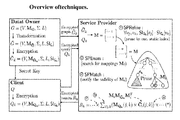•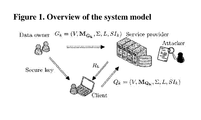•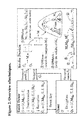•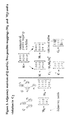•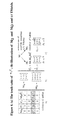•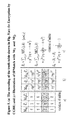•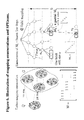•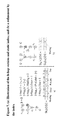•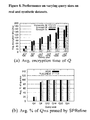•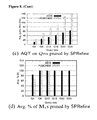•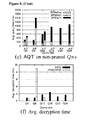•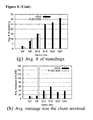•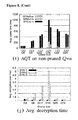•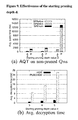•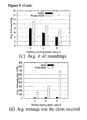•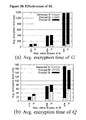•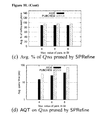•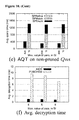•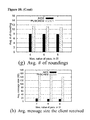•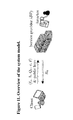•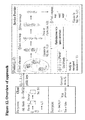•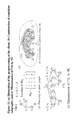•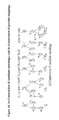•••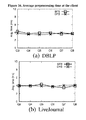•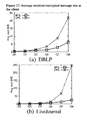••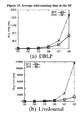•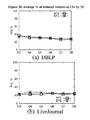•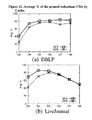•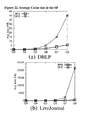•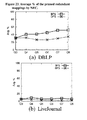•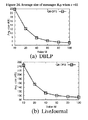## Classifications

• GPHYSICS
• G06COMPUTING; CALCULATING; COUNTING
• G06FELECTRIC DIGITAL DATA PROCESSING
• G06F12/00Accessing, addressing or allocating within memory systems or architectures
• G06F12/1408Protection against unauthorised use of memory or access to memory by using cryptography
• GPHYSICS
• G06COMPUTING; CALCULATING; COUNTING
• G06FELECTRIC DIGITAL DATA PROCESSING
• G06F16/00Information retrieval; Database structures therefor; File system structures therefor
• G06F16/20Information retrieval; Database structures therefor; File system structures therefor of structured data, e.g. relational data
• G06F16/24Querying
• G06F16/245Query processing
• G06F16/2453Query optimisation
• G06F17/30442
• GPHYSICS
• G06COMPUTING; CALCULATING; COUNTING
• G06FELECTRIC DIGITAL DATA PROCESSING
• G06F21/00Security arrangements for protecting computers, components thereof, programs or data against unauthorised activity
• G06F21/60Protecting data
• G06F21/62Protecting access to data via a platform, e.g. using keys or access control rules
• G06F21/6218Protecting access to data via a platform, e.g. using keys or access control rules to a system of files or objects, e.g. local or distributed file system or database
• G06F21/6227Protecting access to data via a platform, e.g. using keys or access control rules to a system of files or objects, e.g. local or distributed file system or database where protection concerns the structure of data, e.g. records, types, queries
• GPHYSICS
• G06COMPUTING; CALCULATING; COUNTING
• G06FELECTRIC DIGITAL DATA PROCESSING
• G06F21/00Security arrangements for protecting computers, components thereof, programs or data against unauthorised activity
• G06F21/60Protecting data
• G06F21/62Protecting access to data via a platform, e.g. using keys or access control rules
• G06F21/6218Protecting access to data via a platform, e.g. using keys or access control rules to a system of files or objects, e.g. local or distributed file system or database
• G06F21/6245Protecting personal data, e.g. for financial or medical purposes
• HELECTRICITY
• H04ELECTRIC COMMUNICATION TECHNIQUE
• H04LTRANSMISSION OF DIGITAL INFORMATION, e.g. TELEGRAPHIC COMMUNICATION
• H04L9/00Cryptographic mechanisms or cryptographic arrangements for secret or secure communication
• H04L9/008Cryptographic mechanisms or cryptographic arrangements for secret or secure communication involving homomorphic encryption
• HELECTRICITY
• H04ELECTRIC COMMUNICATION TECHNIQUE
• H04LTRANSMISSION OF DIGITAL INFORMATION, e.g. TELEGRAPHIC COMMUNICATION
• H04L9/00Cryptographic mechanisms or cryptographic arrangements for secret or secure communication
• H04L9/30Public key, i.e. encryption algorithm being computationally infeasible to invert or user's encryption keys not requiring secrecy
• H04L9/3066Public key, i.e. encryption algorithm being computationally infeasible to invert or user's encryption keys not requiring secrecy involving algebraic varieties, e.g. elliptic or hyper-elliptic curves
• GPHYSICS
• G06COMPUTING; CALCULATING; COUNTING
• G06FELECTRIC DIGITAL DATA PROCESSING
• G06F2212/00Indexing scheme relating to accessing, addressing or allocation within memory systems or architectures
• G06F2212/10Providing a specific technical effect
• G06F2212/1052Security improvement

## Abstract

The present invention relates to solving the issues related to subgraph query services with tunable preservation of privacy of structural information. More particularly, it relates to a novel cyclic group based encryption (CGBE) method for private matrix operations.

## Description

CROSS REFERENCE

This application claims the benefit of U.S. 62/170,122 filed Jun. 2, 2015. The entire disclosure of the preceding application is hereby incorporated by reference into this application.

FIELD OF INVENTION

The present invention relates to solving the issues related to subgraph query services with tunable preservation of privacy of structural information. More particularly, it relates to a novel cyclic group based encryption (CGBE) method for private matrix operations.

BACKGROUND OF INVENTION

Graphs are powerful tools for a wide range of real applications, from biological and chemical databases, social networks, citation networks to information networks. Large graph data repositories have been consistently found in recent applications. For example, PubChem is a real database of chemical molecules, which can be freely accessed via its web interface, for its clients to query chemical compounds. Another example, namely Daylight, delivers chemistry and chemical informatics technologies to life science companies and recently, it has provided web services to allow clients to access its technologies via a network. Subgraph query (via subgraph isomorphism), which is a fundamental and powerful query in various real graph applications, has actively been investigated for performance enhancements recently. However, due to the high complexity of subgraph query, hosting efficient subgraph query services has been a technically challenging task, because the owners of graph data may not always possess the information technology (IT) expertise to offer such services and hence may outsource to query service providers (SP). SPs are often equipped with high performance computing utilities (e.g., a cloud) that offer better scalability, elasticity and IT management. Unfortunately, as SPs may not always be trusted, security (such as the confidentiality of messages exchanged) has been recognized as one of the critical attributes of Quality of Services (QoS). This directly influences the willingness of both data owners and query clients to use SP's services. In the past decade, there is a bloom on the research on query processing with privacy preservation, for example, in the context of relational databases, spatial databases and graph databases. However, up to date, the problem of efficient private subgraph query has not yet been solved.

Therefore, it is an objective of the present invention is to provide solution to structure-preserving subgraph queries that is both efficient and private. The present invention solves structure preservations at two levels. The first level is termed structure-preserving subgraph query processing (SPQP) which preserves the structures of both subgraph queries and data graphs. The second level is termed asymmetric structure-preserving subgraph query processing (ASPQP) which preserves only the structure of subgraph queries. For ease of exposition, one shall present the present invention for SPQP and that for ASPQP separately.

Citation or identification of any reference in this section or any other section of this application shall not be construed as an admission that such reference is available as prior art for the present application.

SUMMARY OF INVENTION

Accordingly, it is an object of the present invention to provide in a first aspect of the present invention a method for structure-preserving subgraph query processing comprising operations of

providing for a modified Ullmann's algorithm to create a structure-preserving structure;

facilitating structure-preserving structure computations;

providing private computations in encrypted domains for the structure-preserving structure computations; and

optimizing mapping enumerations to correctly determine the subgraph structures.

The method according to the first aspect of the present invention wherein the modified Ullmann's algorithm comprising steps of

enumerating all possible subgraph isomorphism mappings Mis from query graph Q to data graph G;

verifying if the mapping Mi is valid or not; and

reducing the search space of Mis by degree and neighborhood constraints.

The method according to the first aspect of the present invention wherein the structure-preserving structure computations comprising steps of

transforming the structure-preserving structure into a series of mathematical computations via operations comprising

enumerating all Mis;

verifying the validity of Mi by additions and multiplications using adjacency matrices of query MQ and M G , where M G is the complement of adjacency matrices of graph MG; and

reducing the search space of Mis by inner products using static indexes SIQ and SIG of Q and G, wherein SIQ (SIG) is an ensemble of h-hop information of each vertex of Q (SIG) represented by a bit vector.

The method according to the first aspect of the present invention wherein the private computations are provided via steps comprising using a cyclic group based private-key encryption scheme to encrypt MQ and M G as encrypted matrices of query MQk and encrypted matrices of graph M Gk;

checking the validity of each mapping Mi, under the cyclic group based private-key encryption scheme with negligible false positives; and

aggregating the computational results under the cyclic group based private-key encryption scheme to reduce communication overheads between client and service provider.

The method according to the first aspect of the present invention wherein the optimized mapping enumerations are provided via steps comprising

introducing a protocol that involves the client's participation, who informs service provider useless enumerations;

exploiting private inner products on the static indexes to derive a refinement that reduces the number of possible mappings; and

computing and encrypting indexes of the graphs offline, wherein those of the queries are computed once by clients online.

The method according to the first aspect of the present invention wherein the structure-preserving subgraph query processing is applied to asymmetric structure preserving query service via operations comprising

a candidate subgraph exploration in the absence of query structure, to reduce a large data graph for query processing;

reducing the size of candidate subgraphs by using neighborhood containment;

a subgraph cache to prune the candidate matchings that are enumerated;

a robust encoding scheme and its verification method; and

a model for the client to determine a proper encoding for his/her query.

Accordingly, it is an object of the present invention to provide in a second aspect of the present invention an apparatus for implementing structure-preserving subgraph query processing according to the first aspect of the present invention comprising

modules for a modified Ullmann's algorithm to create a structure-preserving structure;

modules for facilitating structure-preserving structure computations;

modules for providing private computations in encrypted domains for the structure-preserving structure computations; and

modules for optimizing mapping enumerations to correctly determine the subgraph structures.

The apparatus according to the second aspect of the present invention wherein the modified Ullmann's algorithm comprising steps of

enumerating all possible subgraph isomorphism mappings Mis from query graph Q to data graph G;

verifying if the mapping Mi is valid or not; and

reducing the search space of Mis by degree and neighborhood constraints.

The apparatus according to the second aspect of the present invention wherein the structure-preserving structure computations comprising steps of

transforming the structure-preserving structure into a series of mathematical computations via operations comprising

enumerating all Mis;

verifying the validity of Mi by additions and multiplications using MQ and M G , where M G is the complement of MG; and

reducing the search space of Mis by inner products using static indexes SIQ and SIG of Q and G, wherein SIQ (SIG) is an ensemble of h-hop information of each vertex of Q (SIG) represented by a bit vector.

The apparatus according to the second aspect of the present invention wherein the private computations are provided via steps comprising

using a cyclic group based private-key encryption scheme to encrypt MQ and M G as MQk and M Gk;

checking the validity of each mapping Mi, under the cyclic group based private-key encryption scheme with negligible false positives; and

aggregating the computational results under the cyclic group based private-key encryption scheme to reduce communication overheads between client and service provider.

The apparatus according to the second aspect of the present invention wherein the optimized mapping enumerations are provided via steps comprising

introducing a protocol that involves the client's participation, who informs service provider useless enumerations;

exploiting private inner products on the static indexes to derive a refinement that reduces the number of possible mappings; and

computing and encrypting indexes of the graphs offline, wherein those of the queries are computed once by clients online.

The apparatus according to the second aspect of the present invention wherein the structure-preserving subgraph query processing is applied to asymmetric structure preserving query service via operations comprising

a candidate subgraph exploration in the absence of query structure, to reduce a large data graph for query processing;

reducing the size of candidate subgraphs by using neighborhood containment;

a subgraph cache to prune the candidate matchings that are enumerated;

a robust encoding scheme and its verification method; and

a model for the client to determine a proper encoding for his/her query.

The apparatus according to the second aspect of the present invention wherein the modules are implemented in software on one or more general purpose microprocessors.

The apparatus according to the second aspect of the present invention wherein the modules are implemented in hardware computer logics.

The apparatus according to the second aspect of the present invention wherein the modules are implemented in one or more sub-apparatus on the same device.

The apparatus according to the second aspect of the present invention wherein the modules are implemented in one or more sub-apparatus across at least one network.

The apparatus according to the second aspect of the present invention wherein the modules are implemented in mobile devices.

The apparatus according to the second aspect of the present invention wherein the modules are implemented in client-server devices.

The apparatus according to the second aspect of the present invention wherein the modules are implemented in peer-to-peer devices.

The apparatus according to the second aspect of the present invention wherein the modules are implemented in both software and hardware.

Those skilled in the art will appreciate that the invention described herein is susceptible to variations and modifications other than those specifically described.

The invention includes all such variation and modifications. The invention also includes all of the steps and features referred to or indicated in the specification, individually or collectively, and any and all combinations or any two or more of the steps or features.

Throughout this specification, unless the context requires otherwise, the word “comprise” or variations such as “comprises” or “comprising”, will be understood to imply the inclusion of a stated integer or group of integers but not the exclusion of any other integer or group of integers. It is also noted that in this disclosure and particularly in the claims and/or paragraphs, terms such as “comprises”, “comprised”, “comprising” and the like can have the meaning attributed to it in U.S. Patent law; e.g., they can mean “includes”, “included”, “including”, and the like; and that terms such as “consisting essentially of” and “consists essentially of” have the meaning ascribed to them in U.S. Patent law, e.g., they allow for elements not explicitly recited, but exclude elements that are found in the prior art or that affect a basic or novel characteristic of the invention.

Furthermore, throughout the specification and claims, unless the context requires otherwise, the word “include” or variations such as “includes” or “including”, will be understood to imply the inclusion of a stated integer or group of integers but not the exclusion of any other integer or group of integers.

Other definitions for selected terms used herein may be found within the detailed description of the invention and apply throughout. Unless otherwise defined, all other technical terms used herein have the same meaning as commonly understood to one of ordinary skill in the art to which the invention belongs.

Other aspects and advantages of the invention will be apparent to those skilled in the art from a review of the ensuing description.

BRIEF DESCRIPTION OF DRAWINGS

The above and other objects and features of the present invention will become apparent from the following description of the invention, when taken in conjunction with the accompanying drawings, in which:

FIG. 1 shows an overview of the system model.

FIG. 2 shows an overview of an embodiment of the present invention.

FIG. 3 shows an adjacency matrices of Q and G; Two possible mappings (M1 and M2) and a violation in C2.

FIG. 4 shows (a) The truth table of MQ Ci ; (b) Illustration of MQ and M G ; and (c) TMatch.

FIG. 5 shows (a) The encoding of the truth table shown; (b) Encryption by CGBE; and (c) Illustration of SPMatch with M1 and M2.

FIG. 6 shows illustration of mapping enumerations and SPEnum.

FIG. 7 shows (a) Illustration of the h-hop vertices and static index; and (b) a refinement by the index.

FIG. 8. Performance on varying query sizes on real and synthetic datasets.

FIG. 9 shows the effectiveness of the starting pruning depth d.

FIG. 10 shows the effectiveness of SI.

FIG. 11 shows an overview of the asynchronous system model.

FIG. 12 shows an overview of the approach.

FIG. 13 shows (a) Illustration of the preprocessing at the client; (b) Construction of candidate subgraphs; and (c) Minimization of CSs by NC.

FIG. 14 shows (a) Construction of candidate matchings; and (b) Enumeration of possible mappings.

FIG. 15 shows SPVerify (and SPVerify*) between Qk and CMs1

FIG. 16 shows the average preprocessing time at the client.

FIG. 17 shows the average received encrypted message size at the client.

FIG. 18 shows the average decryption time at the client.

FIG. 19 shows the average total running time at the SP.

FIG. 20 shows the average % of reduced vertices in CSs by NC.

FIG. 21 shows the average % of the pruned redundant CMs by Cache.

FIG. 22 shows the average Cache size at SP.

FIG. 23 shows the average % of the pruned redundant mappings by NEC.

FIG. 24 shows the average size of messages Rks when c=11.

DETAILED DESCRIPTION OF INVENTION

The present invention is not to be limited in scope by any of the specific embodiments described herein. The following embodiments are presented for exemplification only.

Motivating Example

Consider a pharmaceutical company with revenue that depends mostly on the invention of health care products. The company may have discovered new compounds for a new product. To save laboratory work, it may query the compounds from proprietary biological pathway networks to check whether it is possible for the ingredient compounds to form other compounds via certain chemical reactions (a structural pattern from the network). However, on the one hand, the company is reluctant to expose the queries (the ingredients) to the SP, as it may apply for patents for the synthesis. On the other hand, the owner of the pathway networks may not only lack the expertise to host query services but may also be reluctant to release the networks to the public. The owner is willing to release it to paid users only. Hence, it is crucial to protect both the queries and the network from the SP. Such privacy concerns also arise from social networks and biological networks, among many other applications.

In the present invention, one investigates that the query client may prefer not to expose the structure of query graphs to the SP, and meanwhile, the data owner may not want the SP to be able to infer the structure of their graph data. The fundamental problem being studied is to evaluate subgraph query at the SP with a preservation of the structures of both the query graphs and graph data in the paradigm of the query services. In the present invention, in particular, aims to protect the adjacency matrices of the queries and/or data graph from the SP. To the knowledge, such a problem has never been addressed before.

In the recent work, one has addressed the authenticity of the answers of subgraph query, but not their confidentiality. A host of related work is also on privacy-preserving graph query. However, none of these studies can support subgraph query with the structure preservation of the query and graph data. Another category of related research is on the study of privacy-preserving graph publication. As the published data are modified in a non-trivial manner (e.g., by sanitization), it is not clear how subgraph query can be supported.

The intrinsic difficulty of this problem is that the SP cannot optimize query processing by directly using the structures of the queries and/or data graph, since such information cannot be exposed. However, most of the existing subgraph isomorphism algorithms (e.g., VF2, QuickSI and Turboiso) for the query services must traverse the graph, which by definition leaks structural information. A naïve method is to transfer the entire database to the client for query processing. However, it is inefficient when the database is large and can be infeasible due to the ownership restrictions of the databases.

Our techniques for a structure-preserving subIso (denoted as SPsubIso) are derived from the Ullmann's algorithm, a seminal algorithm for subgraph isomorphism. One revises the Ullmann's algorithm into three steps that form the foundation of the techniques. (1) Enum enumerates all possible subgraph isomorphism mappings Mis from query graph Q to data graph G; (2) Match verifies if the mapping Mi is valid or not; and (3) Refine reduces the search space of Mis by degree and neighborhood constraints. The benefits of adopting the Ullmann's algorithm are twofold: (1) the query evaluation between Q and G is mostly a series of matrix operations between their adjacency matrices MQ and MG. It does not require traversals on structures; and (2) its query evaluation requires simple structures. This makes the privacy analysis simpler.

Specifically, to facilitate structure-preserving computations, one first transforms subIso into a series of mathematical computations, denoted as TsubIso. TsubIso comprises three steps, corresponding to subIso: (1) TEnum enumerates all Mis; (2) TMatch verifies the validity of Mi by additions and multiplications using MQ and M G , where M G is the complement of MG; and (3) TRefine reduces the search space of Mis by inner products on the present static indexes SIQ and SIG of Q and G, where SIQ (SIG) is an ensemble of h-hop information of each vertex of Q (SIG) represented by a bit vector.

The major benefit of these three steps of TsubIso is that only mathematical operations are involved, which allows an adoption of private computations in encrypted domains. Based on TsubIso, one presents the novel structure-preserving subIso (SPsubIso). In particular, one first describes a new private-key encryption scheme, namely cyclic group based encryption scheme (CGBE), to encrypt MQ and M G as MQk and M Gk. Then, one introduces SPMatch involving the additions and multiplications under CGBE to check the validity of each mapping Mi, with negligible false positives. Further, the computation results under CGBE can be aggregated to reduce communication overheads between the client and the SP. One proves that CGBE is perfectly secure under chosen plaintext attack and the SP cannot learn any structures from SPMatch.

Next, one describes SPEnum which optimizes the mapping enumeration by introducing a protocol that involves the client's participation, who informs the SP useless enumerations. In addition, to optimize SPsubIso, one develops SPRefine which exploits private inner products on the static indexes to derive a refinement that reduces the number of possible mappings. The indexes of the graphs are computed and encrypted offline, whereas those of the queries are computed once by the clients online. One analyzes the effects of these optimizations on the probabilities that the SP may correctly determine graph structures. Therefore, the clients may tune the trade-off between performances and privacy requirements.

To summarize, the contributions of the present invention for structure-preserving subgraph query processing (SPQP) are as follows:

• We transform the Ullmann's algorithm subIso as TsubIso. It only involves a constant number of mathematical computations (with respect to the given data and query sizes), such that its private version can be introduced and analyzed;
• We present a structure-preserving subIso (SPsubIso) based on TsubIso, consisting of SPMatch, SPEnum and SPRefine. Specifically, one describes CGBE for SPMatch, which supports efficient encryption and decryption, partial additions and multiplications, and aggregation of computation results. One introduces a protocol for SPEnum that involves the client to eliminate useless mappings. One describes SPRefine that exploits private inner products of static indexes to further optimization;
• We analyze the privacies of SPMatch, SPEnum and SPRefine; and
• We conduct detailed experiments to verify that SPsubIso is efficient and the optimizations are effective.

Problem Formulation

This section presents a formulation of the problem studied in the present invention for SPQP. More specifically, one presents the system model, privacy target, attack model, and problem statement.

System Model.

One follows the system model that has been well received in the literature of database outsourcing (shown in FIGS. 1 and 2), and known to be suitable for many applications. It consists of three parties:

(1) Data owner: The owner owns and encrypts the graph data G. He/she then outsources the encrypted graph to the service provider and delivers the secret keys to clients for encryption of the query graphs and decryption of the encrypted result;

(2) Service provider (SP): The SP may be equipped with powerful computing utilities such as a cloud. The SP evaluates a client's query over the encrypted data, on behalf of the data owner, and returns the encrypted result to the client; and

(3) Client: A client encrypts the query graph Q using the secret keys, submits it to the SP, and decrypts the returned encrypted result to obtain the final answer.

Attack Model.

One assumes the dominating semi-honest adversary model from literature, where the attackers are honest-but-curious and the SP may also be the attacker. For presentation simplicity, one often terms the attackers as the SP. One assumes that the attackers are the eavesdroppers and adopt the chosen plaintext attack. One assumes that the SP and clients are not allowed to collude.

Privacy Target.

To facilitate a technical discussion, one assumes that the privacy target is to protect the structures of a query graph Q and a graph data G from the SP under the attack model defined above. The structural information of Q and G considered is the adjacency matrices of Q and G, respectively. More specifically, the probability that the SP correctly determines the values of the adjacency matrix of the graph is guaranteed to be lower than a threshold with reference to that of random guess.

The problem statement of the present invention can be stated as follows: Given the above system and attack model, one seeks an efficient approach to facilitate the subgraph isomorphism query services with preserving the above defined privacy target.

Preliminaries

In this section, one first discusses the background for the subgraph query and revise the classical Ullmann's algorithm.

Subgraph Query

The present invention assumes a graph database is a large collection of graphs of modest sizes. One considers undirected labeled connected graphs. A graph is denoted as G=(V,E,Σ,L), where V(G), E(G), Σ(G) and L are the set of vertices, edges, vertex labels and the function that maps a vertex to its label, respectively. One uses Deg(vi,G) to denote the degree of the vertex vi in graph G. In the present invention, one focuses on the graph with only vertex labels. the present techniques can be extended to support the graph with edge labels with minor modifications.

Definition 1:

Given two graphs G=(V,E,Σ,L) and G′=(V′,E′,Σ′,L′), a subgraph isomorphism mapping from G to G′ is an injective function ƒ:V(G)→V(G′) such that
u∈V(G),ƒ(u)∈V(G′),L(u)=L′(ƒ(u)); and
∀(u,v)∈E(G),(ƒ(u),ƒ(v))∈E(G′).

We say a graph G is a subgraph of another graph G′ if and only if there exists a subgraph isomorphism mapping (in short mapping) from G to G′, denoted as GG′ or subIso (G, G′)=true. It is known that deciding whether G is the subgraph of G′ is NP-hard. Subgraph isomorphism query or simply subgraph query can be described as follows.

Definition 2:

Given a query graph Q and a graph database, the subgraph query is to retrieve the graphs from the database where Q is a subgraph of the graphs.

Revised Ullmann's Algorithm

Subgraph query has been a classical query. As motivated in an earlier section, the Ullmann's algorithm is simple for privacy preservation. In this subsection, one revises the Ullmann's algorithm into three interleaving steps, namely enumeration, matching and refinement. These form a foundation of the discussions.

Prior to the algorithmic details, one presents some notations used in the present invention. One uses subIso to refer to as the Ullmann's algorithm. One denotes a query as Q=(V,MQ,Σ,L) and graph as G=(V,MG,Σ,L), m=|V(Q)| and n=|V(G)|, MQ and MG are the adjacency matrices of Q and G, respectively. MQ(j,k) is a binary value, where MQ(j,k)=1 if (vj,vk)∈E(Q), and otherwise 0. The symbols j, k here are node ids (aka node index). For presentation simplicity, One may sometimes use the nodes themselves (i.e. MQ (vi,vj)) to denote the entries of the adjacency matrix when such a notation makes the presentation clearer. In particular, the former is often used for the description of the invention for SPQP and the latter is often adopted for describing that for ASPQP. The values of the entries of MG are defined, similarly. Both adjacency matrices MQ and MG carry the most fundamental structural information, i.e., the edge information. One uses a m×n binary matrix M to represent the vertex label mapping between Q and G. Specifically, ∀j,k, M(j,k)=1 if L(vj)=L(vk), where vj∈V(Q) and vk∈V(G); and otherwise 0.

The revised Ullmann's algorithm (subIso) is detailed in Algo. 1. subIso takes Q and G as input and returns true if Q is the subgraph of G. Initially, it determines the vertex label mapping M (Lines 1-2). Then, subIso checks from M if there is a subgraph isomorphism mapping from Q to G by using three steps: (1) Enum; (2) Match; and (3) Refine. Next, one highlights some details of each step.

Enumeration (Lines 8-17).

Enum enumerates all possible subgraph isomorphism mappings from Q to G by M. Each possible mapping is denoted as Mi. Each column of Mi contains at most one 1 and each row of Mi has only one 1 (Lines 12-13). Mi is enumerated from M row by row (Line 14). When an Mi is obtained (Line 8), Match checks if Mi is a subgraph isomorphism mapping (Line 9). It is easy to see that the number of possible Mis enumerated is O(nm).

Algorithm 1 Revised Ullmann′s algorithm subIso (Q,G) Input: The query graph Q and the data graph G Output: True if Q is a subgraph of G, False otherwise 1: Initialize Mi := 0 2: Generate M from (V,Σ,L) of Q and G 3: if!Refine (M,Q,G)  /* Refinement */ 4:    return False 5: if !Enum (0,Mi,M,Q,G)  /* Enumeration */ 6:    return False 7: return True Procedure 1.1 Enum (d,Mi,M,Q,G) 8: if d = m 9:     return Match (Mi,Q,G)  /* Matching */ 10: if!Refine (M,Q,G)  /* Refinement */ 11:    return False 12: for each c, where c < n,M(d,c) = 1, and ∀d′ < d,Mi(d′,c) = 0 13:    Mi(d,c) := 1 14:    if Enum (d + 1,Mi,M,Q,G) 15:       return True 16:    Mi(d,c) := 0 17: return False Procedure 1.2 Match (Mi,Q,G) 18:  Ci = MiMGMi T      /* violation*/ 19:  if ∃j,k,MQ(j,k) = 1

Ci(j,k) = 0 20:    return False 21:  return True Procedure 1.3 Refine (M,Q,G) 22:  do ∀j,k,M(j,k) = 1 23:      if degree constraint or neighborhood constraint fails 24:        M(j,k) := 0 25:   while M is not changed 26:  if ∃j,s.t.,∀k,M(j,k) = 0 27:      return False 28:  return True

Matching (Lines 18-21).

For each Mi enumerated from M, if there exists a matrix Ci, Ci=MiMGMi T, such that ∃j,k,
M Q(j,k)=1

C i(j,k)=0  (1)
then such an Mi cannot be an subgraph isomorphism mapping from Q to G. Note that Ci intuitively represents the adjacency matrix of a subgraph of G, that Q may be isomorphic to through Mi. Formula 1 states that there is an edge between vertices j and k in Q but no corresponding edge in the subgraph of G, represented by Ci. Such an Mi is definitely not a mapping. One terms the case in Formula 1 as a violation of subgraph isomorphism (or simply violation). Mi without violation is called a valid mapping. That is, Q is a subgraph of G through Mi.

Refinement (Lines 22-28).

The number of l's in M significantly increases the number of Mi to be enumerated in worst case. In the Ullmann's algorithm, there are two optimizations, called refinements, to reduce the number of 1's in M. Intuitively, the first refinement exploits the degree constraint, whereas the second refinement relies on the neighborhood constraint: ∀j,k, M(j,k)=1

Deg(v j ,Q)≤Deg(v k ,G); and  (1)
x,M Q(j,x)=1y,M(x,y)M G(k,y)=1.  (2)

Refinement is performed when (1) M is determined (Line 3) and (2) Mis are enumerated (Line 10). For any pair of j and k, M(j,k)=1, if either one of the constraints is not satisfied, the algorithm then flips M(j,k), i.e., sets M(j,k)=0 (Lines 22-24). If any row of M contains only 0s, it reports there is no valid mapping (Lines 26-27).

Example 1 FIG. 3 Shows an Example for Algo. 1

The LHS shows the query graph Q and the data graph G and their adjacency matrices (below the graphs). The RHS shows the enumeration of Mis. C1 is computed by M1, which is a valid mapping from Q to G. Suppose one does not perform Refine, M2 will be enumerated. Match determines that M2 contains violations, as shown. However, when Refine is performed, M(1,4) is flipped to 0 as v4 of G does not connect to v2 and Deg(v1,Q)>Deg(v4,G). M2 is not enumerated at all.

subIso with Matrix Operations

From subIso in Algo. 1, it can be noted that the violation defined by Formula 1 in Match (Line 19) is determined by processing of the entries between MQ and Ci, and the neighborhood constraint (Line 23) precisely exploits edge information. Hence, as motivated in an earlier section, one casts subIso into an algorithm that uses a series of mathematical computations, denoted as TsubIso. This enables us to derive private versions of such operations in later sections.

Foremost, one extends the definition of the query and data graph (Q and G), defined in . This extended definition only differs from the one presented previously that the entries in the adjacency matrix MG are flipped, i.e., 0s (resp. 1s) are set to 1s (resp. 0s), for the transformed subIso (to be detailed soon). Moreover, Q and G are extended with precomputed indexes, called static indexes, to enhance performances. Since the subsequent discussions always assume the extended queries/graphs, one omits the term “extended” for brevity.

Definition 3:

The extended data graph of G is denoted as G=(V,M G ,Σ,L,SIG) and the query graph is extended as Q=(V,MQ,Σ,L,SIQ), where M G are flipped, i.e., ∀j,k,
M G (j,k)=

M G(j,k)
and SIG and SIQ (called static indexes) are sets of bit vectors, for optimization purposes.

Based on Def. 3, one rewrites subIso into transformed subIso called TsubIso in Algo. 2. The inputs are the query graph Q and data graph G. It returns 0 if Q is a subgraph of G, and non zero otherwise. The corresponding three main steps of Algo. 1 in Algo. 2 are highlighted below.

Transformed Enumeration.

The main difference in TEnum is that Refine (Lines 10-11 of Algo. 1) is removed. The reason is that Refine exploits structural information, which is required to keep private. Another difference is that TEnum is invoked with an input message R that aggregates the subgraph isomorphism information from Q to G during the enumeration of Mis.

Algorithm 2 TsubIso (Q,G) Input: The query graph Q and the transformed data graph G Output: R = 0 if Q is a subgraph of G, R = 1 otherwise 1: Initialize R := 1,Mi := 0 2: Generate M from (V,Σ,L) of Q and G 3: if!Refine (M,Q,G)  /* TRefinement */ 4:   return R 5: TEnum (0,Mi,M,Q,G,R)  /* TEnumeration */ 6: return R Procedure 2.1 TEnum (d,Mi,M,Q,G,R) 7: if d = m 8:   TMatch (Mi,Q,G,R)  /* TMatching */ 9: for each c, where c < n,M(d,c) = 1, and ∀d′ < d,Mi(d′,c) = 0 10:   Mi(d,c) := 1 11:   TEnum (d + 1,Mi,M,Q,G,R) 12:   Mi(d,c) := 0 Procedure 2.2 TMatch (Mi,Q,G,R) 13:  Initialize Ri := 0,MCi := 0 14: C l = MiM G Mi T 15:  ∀j,k,MCi(j,k) := MQ(j,k) × C l(j,k)  /* Multiplication */  16:  Ri := Σ∀j,kMCi(j,k)  /* Addition */ 17:  R ×= Ri /* Multiplication */ Procedure 2.3 TRefine (M,Q,G) 18:  for each j,k,M(j,k) = 1 19:    if SIQ[vi] · SIQ[vj] ≠ SIQ[vj] · SIG[vk] 20:     M(j,k) = 0 21:  if ∃j,s.t.,∀k,M(j,k) = 0 22:    return False 23:  return True

Transformed Matching.

In Match, the violation of Formula 1 (Line 19 of Algo 1) is checked by a condition defined on each entry of MQ and Ci, which leaks structural information. In comparison, with Def. 3, the presence of a violation is detected from the product of the matrices MQ and Ci (Lines 14-15 of Algo 2) in TMatch. Further, the violation due to Mi is preserved under aggregations, i.e., the result of Mi (denoted as Ri) is aggregated into one message R (Lines 16-17). The detection of a violation in TMatch is illustrated with FIG. 4. Similar to Match, TMatch computes the “subgraph” Ci that Q may be isomorphic to. With the data graph, Ci is computed in Line 14. There are four possible cases of the entries of MQ and Ci and FIG. 4 a) highlights the case of the violation of Formula 1. That is, ∃j, k, MQ(j,k)=1 and Ci(j,k)=0 (thus, Ci (j,k)=1), then
M Q(j,k) C i (j,k)=1  (2)

For the other three cases, the product is 0. Therefore, by Formula 2, TMatch detects the violation and aggregates the results as follows:

1. Multiplication (Line 15). For each pair of (j,k), TMatch computes MCi(j,k)=MQ(j,k)×Ci(j,k);

2. Addition (Line 16). TMatch sums up the entries of the product MCi, i.e., Ri∀j,k MCi(j,k). Note that Ri intuitively represents the validity of the mapping Mi, i.e., if Mi is valid, no violation is found and the value of Ri is 0, by Formula 2; and

3. Multiplication (Line 17). TMatch then aggregates Ri into R by a multiplication, i.e., R=R×Ri. If there is at least a valid Mi, the value of R equals 0, and non zero otherwise.

It is worth highlighting that if there exists a subgraph isomorphism mapping Mi from Q to G, then Mi contains no violation, R=0 and R=0. Thus, R=0 implies that Q is a subgraph of G. Otherwise, R is non zero, which implies all Ris are not zero and there must be some 1's in the entries of MCi, for all i. By Formula 2, there is a violation in each Mi and thus, Q is not a subgraph of G.

Example 2

One illustrates TMatch with the example shown in FIGS. 4 b) and c). The query and graph are those shown in FIG. 4. FIG. 4 b) presents MQ and M G . FIG. 4 c) reports the intermediate results of TMatch of two possible mappings M1 and M2 (FIG. 3). M1 is a valid mapping as R1 computed using MQ and C1 (in Lines 15-16) is 0. In comparison, R2 computed using MQ and C2 is 2. Hence, M2 is an invalid mapping. R=R1×R2=0 indicates that there is a valid mapping and thus Q is a subgraph of G.

Transformed Refinement.

As the neighborhood constraint of Refine precisely exploits the edge information, it cannot be directly adopted. One transforms Refine as TRefine that inner products (Line 19) between the present static index (SI, in the form of bit vector) are used for refinements. The index is called static as the indexes of the data graphs are precomputed and those of query graphs are computed by the client prior to TsubIso.

It is worth noting that TsubIso is mainly a series of mathematical operations, i.e., additions, multiplications and inner products. This enables us to establish a connection to private query processing.

Structure-Preserving subIso

In this section, one describes structure-preserving subIso, denoted as SPsubIso (recall in FIG. 2). SPsubIso contains three steps: (1) structure-preserving Match (SPMatch); (2) structure-preserving Enum (SPEnum); and (3) structure-preserving Refine (SPRefine).

Before presenting the details, one first gives the definition of the encrypted query graph Qk and the transformed graph G k, which are shared by SPMatch, SPEnum and SPRefine.

Definition 4:

The encrypted Q and G are denoted as Qk and G k, respectively, where Qk=(V,MQk,Σ,L,SIQ k ) and G k=(V,M Gk,Σ,L,SIG k ). MQk (M Gk) and SIQ k (SIQ) are the encrypted MQ (M G ) and SIQ (SIG), respectively.

It is worth remarking that one only protects MQ (resp. M G ) and SIQ (resp. SIG) in Q (resp. G), by using encryption, since (V,Σ,L) does not expose the structural information.

Structure-Preserving Matching

In this subsection, one adopts cyclic group and describe a novel private-key encryption scheme to encrypt MQ and M G . One then presents SPMatch to compute the operations of TMatch in encrypted domain, where the mapping (Mi) has been enumerated by SPEnum.

Cyclic Group Based Encryption

Recall that TMatch involves both additions and multiplications. Hence, the state-of-the-art partially homomorphic encryption schemes (e.g., Paillier and ElGamal) cannot be adopted to the problem. On the other hand, due to the known performance concerns of fully homomorphic encryption scheme (FHE), one may not directly adopt FHE either.

Therefore, one presents a private-key encryption scheme, namely cyclic graph based encryption scheme (CGBE). CGBE not only supports both partial additions and multiplications, but also allows efficient encryption and decryption. Importantly, it is secure against CPA. However, the trade-off of using CGBE in SPMatch is that (1) it introduces negligible false positives; and (2) it requires multiple encrypted messages for aggregating a query result, which are sent to the client.

Before the detailed discussion, one first present the preliminary about cyclic group. Let G be a group. p=|G| is denoted as the order of G. In particular, ∀g∈G, the order of G is the smallest positive integer p such that gp=1. Let (g)={gi:i∈Zp,gi∈Zn}={g0, g1, . . . , gp-1} denote the set of group elements generated by g. The group G is called cyclic if there exists an element g∈G such that <g>=G. In this case, the order of G is p=|G| and g is called a generator of G. Next one presents the cyclic group based encryption scheme as follows.

Definition 5:

The cyclic group based encryption scheme is a private-key encryption scheme, denoted as CGBE=(Gen, Enc, Dec), where

• Gen is a key generation function, which generates a secrete key x∈[0,p−1] uniformly at random, a cyclic group <g>={gi:i∈Zp,gi∈Zn}. It outputs the private keys as (x, g) and the value p which is known to the public.
• Enc is an encryption function, which takes as input a message m and the secrete key (x, g). It chooses a random value r, and outputs the ciphertext
c=mrg x(mod p)
• Dec is a decryption function, which takes as input a ciphertext c, and the secrete key (x, g).
It outputs
mr=cg −x(mod p)
Note that the Dec function of CGBE only decrypts the ciphertext c as the product of the message m and random value r. This is because SPMatch does not require the exact value of m.

Encryption of MQ and MG

To encrypt MQ and M G , one first presents an encoding for each entry of MQ and M G .

Definition 6:

The encoding of the entries of MQ and M G are: ∀j,k,
if M Q(j,k)=0, set M Q(j,k) as q; and
if M G (j,k)=0, set M G (j,k) as q,
where q is a large prime number.

In relation to Def. 6, one has the following Formula 3 that is similar to Formula 2 to detect the violation. One notes that only in case of MQ(j,k)=1 and Ci(j,k)=1,
M Q(j,k C i(j,k)=1(mod q),  (3)
where Ci=MiM G Mi T. the product will be 0 otherwise. FIG. 5 a) shows the encoding of four possible combinations between entries, one can see that only if MQ(j,k)=1 and Ci(j,k)=1, the product becomes 1. Otherwise it is 0.

Under the encryption scheme CGBE in Def. 5 and the encoding in Def. 6, one is ready to define the encryption of the encoding of MQ and M G (in short, the encryption of MQ and M G ) as follows.

Definition 7:

The encryption of MQ and M G are denoted as MQk and M Gk, respectively, where ∀j,k,
M Qk(j,k)=Enc(M Q(j,k),x,g)
M Gk(j,k)=Enc(M G (j,k),x,g)  (4)

Example 3

One uses FIG. 5 b) to illustrate an example of the encryption of MQ by CGBE. ∀j,k, if MQ(j,k)=1, MQk(j,k)=Enc(1,x,g)=rgx (mod) p; and if MQ(j,k)=q, MQk(j,k)=Enc(q,x,g)=qrgx (mod) p.

Finally, one remarks that the large prime number q for the encoding (Def. 6) must be kept secret. Since CGBE is a symmetric encryption scheme, both the DO and the client hold the same keys (x,g,p), whereas SP keeps p only.

SPMatching

Based on Def. 7, one presents a cyclic group based matching (in short, SPMatch) derived from TMatch (in Algo. 2), shown in Algo. 3. In particular, the input value Rk is the encrypted message that aggregates the violation. SPMatch first generates Ci (Line 1), which is computed from Mi and M Gk. Then the following three steps are invoked.

Algorithm 3 SPMatch (Mi,Qk,G k,Rk) 1: C l := MiM G k Mi T   /* Multiplication */ 2: ∀j,k,MCi(j,k) := MQ k (j,k) × C l(j,k) (mod p)   /* Addition */ 3: Ri := Σ∀j,kMCi(j,k) (mod p) 4: if i ≠ 0,i mod ω ≠ 0  /* Multiplication */ 5:    Rk ×= Ri (mod p) 6: else Send Rk to client, Rk := Ri

1. Multiplication (Line 2). For each pair of (j,k), SPMatch computes MCi(j,k)=MQk(j,k)×Ci(j,k) (mod p);
2. Addition (Line 3). SPMatch sums up the entries in the product, i.e., Ri∀j,k MCi(j,k) (mod p). If Mi is valid, i.e., no violation is found, the decryption of the sum is exactly 0, by Formula 3; and
3. Multiplication (Lines 4-6). SPMatch then aggregates Ri into Rk by multiplication (Line 5). If there is at least one valid mapping from Q to G, the decryption of a Rk equals 0. Otherwise, the decryption value is non zero. One remarks that CGBE leads to errors if the number of Ris in Rk is larger than a predetermined value w. One thereby presents a decomposition scheme (discussed later) that sends to the client a sequence of Rks, where each Rk aggregates ω Ri (Line 4).

Example 4

FIG. 5 b) shows an example to illustrate the multiplication of the four possible cases of combinations between MQk and Ci . One observes that only under the violation (shown in grey shadow), the product of MQk and Ci does not contain q. FIG. 5 c) illustrates an example of SPMatch following FIG. 4 c). R1 and R2 are computed by the summations of MC1 and MC2, respectively. Note that R2 contains violation as M2 is not a valid mapping. Rk is produced.

Decryption at the Client.

After receiving all the encrypted results Rk, the client performs the decryption, which mainly contains two steps as below.

1. For each message Rk aggregated with ω Ris, the client computes the message encoded in Rk as Rk′=Dec(Rk,x,g)); and

2. For each encoded message Rk′, the client computes the final result by R=Rk′ mod q.

If any of R equals to 0, there is at least one valid isomorphic mapping Mi that contributes a 0 (Line 3) to the product Rk (Lines 4-5). Thus subIso(Q,G)=true.

Example 5

One shows the decryption at client following FIG. 5 c). The encrypted message Rk client receives aggregates two Ris. The client first generates (g−x)2×2, computes Rk′=Rk×g−4x (mod p), and finally computes R=Rk′ mod q. The result is 0 that indicates Q is a subgraph of G.

Decomposition Scheme.

Once the number of Ri aggregated by Rk exceeds a predetermined value, SPMatch will result in incorrect answer. The reason leading to this problem is the multiplications when aggregating Ri into Rk in Line 5 of Algo. 3. Recall that in the decryption, the client needs to compute the encoded message Rk′ after receiving Rk, once Rk′ exceeds p, the client can never recover the final result R by modular q correctly. One can overcome this limitation by determining the maximum number of Ris that can be aggregated in Rk, denoted as ω. One has the following formula:

$Len ⁡ ( R i ′ ) = 2 × ( Len ⁡ ( q ) + Len ⁡ ( r ) ) + log ⁡ ( m 2 ) ⁢ ⁢ Len ⁡ ( p ) ≥ ω × Len ⁡ ( R i ′ ⁢ ) ⁢ ⇔ ω ≤ Len ⁡ ( p ) Len ⁡ ( R i ′ ) ( 5 )$
where m=|V(Q)|, Len(x) is the size of the value x, and Ri′ is the message encoded in Ri, i.e., Ri′=Dec(Ri,x,g)2. In particular, with reference to Algo. 3, (Len(q)+Len(r)) is the largest size of the message encoded in each entry of MQk and Ci. The size of their product (Line 2) is 2(Len(q)+Len(r)). There are m2 additions of such products (Line 3), hence, Algo. 3 requires at most log(m2) carry bits. This gives us the largest size of an Ri′. Then, the size of ωRi′ values must be smaller than that of p, and one obtains the inequality in Formula 5. Having computed w, the SP decomposes Rk into a number of aggregated messages, each of which is a product of at most ω Ris.

False Positive.

When performing SPMatch, one finds that two operations introduce false positives: (1) additions with computing Ri (Line 3); and (2) multiplications with computing Rk in each decomposition (Line 5). One proves that the probabilities of the above two false positive are negligible. Next, one first analyzes the probability of false positive from the additions with computing Ri.

Proposition 1:

The probability of false positive in Ri is negligible.

Proof.

The probability of false positive in Ri is

$Pr ⁡ ( false ⁢ ⁢ positive ⁢ ⁢ in ⁢ ⁢ R i ) = Pr ⁡ ( r 1 + … + r m 2 = 0 ) ⁢ ( mod ⁢ ⁢ q ) = 1 q . ( 6 )$
where m=V(Q), and q is a large prime number, e.g., 32 bits. Thus, the probability is negligible in practice.

Proof:

The probability of false positive in Ri is

$Pr ⁡ ( false ⁢ ⁢ positive ⁢ ⁢ in ⁢ ⁢ R i ) = Pr ⁡ ( r 1 + … + r m 2 = 0 ⁢ ( mod ⁢ ⁢ q ) ) = 1 q , ( 6 )$
where m=V(Q), and q is a large prime number, e.g., 32 bits.
Thus, the probability is negligible in practice.
Based on Prop. 1, one is able to analyze the probability of false positive with computing the Rk in each decomposition.

Proposition 2:

The probability of false positive in Rk is negligible in each decomposition.

Proof.

The probability of false positive in each Rk is

$Pr ⁡ ( falsepositiveR k ) = ⁢ Pr ⁡ ( falsepositiveinallitsR i ) = ⁢ 1 - ( 1 - 1 q ) ω ≈ ⁢ 1 - e - ω q ; ( 7 )$
where ω is the size of the decomposition. Since ω<<q, the probability is negligible in practice.

Structure-Preserving Enumeration

The mappings (Mis) processed by SPMatch are enumerated by SPEnum. Since the worst case number of all possible mappings Mis from M (Lines 7-12, Algo. 2) is O(nm), it has been a crucial task of SPsubIso to prune the search of useless Mis. For instance, one shows a scenario of useless enumerations by using the LHS of FIG. 6. There are four subgraphs of G in grey, which are disconnected from each other. In the example, only 4 mappings out of 46 are possible and the remaining enumerated mappings are useless. However, since both G and Q are encrypted, the SP can only blindly enumerates those mappings even they may appear “certainly” invalid.

Therefore, one presents SPEnum that consists of a protocol between the SP and the client to prune some useless partial mappings. However, due to the pruned enumerations, a little non-trivial structural information may be leaked. Such information leakage can be well controlled by determining how often the client informs the pruning.

Mapping Enumeration as a Search Tree

To facilitate the discussions on pruning, one views the search of possible subgraph isomorphic mappings from Q to G (in the LHS of FIG. 6) as a search tree, as in the literature of optimizations. A sketch is shown in the RHS of FIG. 6. Each internal node in the d-th level represents a partial mapping Mi, denoted as Mi′, whose enumeration is only up to the first d rows of M. One denoted Q′ as the induced subgraph of Q from the first d vertices of Q and G′ as the subgraph that Q′ maps to, under Mi′. In the example, the query size is 6, thus the height of the search tree is 6. The fanout of each internal node in d-th level equals to the number of 1s in the (d+1)-th row of M. Each leaf node of the search tree represents a complete Mi. Without pruning, TEnum enumerates all 46 leaf nodes.

SPEnumeration

We next present SPEnum. It adopts TEnum and SPMatch, and determines if a partial mapping Mi′ between Qk′ and G k′ is a valid to be expanded or not. The inputs of SPMatch are (1) Mi′, which is the current partial mapping in d-th level; (2) Qk′ and G k′, which are the induced subgraph of Qk and G k with the first d vertices, respectively; and (3) Rk, which is the same as before. Then, a naive protocol involving the client is described as follows:

1. SP enumerates an Mi′ and performs SPMatch. Its result Rk is transmitted to the client for decryption immediately.

2. The client decrypts Rk. If it is 0, Qk′ is subgraph isomorphic to G k′ and Mi′ is valid. The client then notifies the SP to repeat Step 1 to continue the enumeration from Mi′. Otherwise, the search subtree at Mi′ can be pruned.

3. SP terminates SPEnum when a valid Mi is found or no more Mis can be enumerated.

Protocol with Aggregated Messages.

The naive protocol introduces additional network communications between the client and the SP. To control such communication overhead, one adopts a breath first search (BFS) to TEnum and exploit the aggregation supported by CGBE. Specifically, suppose SPEnum is at the d-th level of the search tree, it conducts two rounds of communications.

1. In the first round, for each internal nodes n with a valid partial mapping at the d-th level (e.g., n1 and n4 at the 3rd level in FIG. 6), the SP processes its child nodes of n in batches of the size ω. For each batch, the SP performs SPMatch at each node and aggregates ω Ris into Rk. Recall that ω is the number of messages that CGBE correctly aggregates. The SP sends all the Rks to the client.
The client decrypts all Rks. (i) If it is 0, there exists at least one child node n′ of n such that its partial mapping is valid (e.g., n1). (ii) Otherwise, there is no valid partial mapping of the n's child nodes. The search subtree of n can be safely pruned (e.g., n4).
2. In the second round, the SP then sends all Ris of the child nodes of each batch that contains valid partial mappings to the client, and determines at which node(s) the search proceeds (e.g., n2). Step 1 is then repeated at the (d+1)-th level (e.g., at the 4th level).
3. SP terminates SPEnum as in the naive protocol.

Remarks.

It is worth noting that CGBE limits ω Ri to be aggregated into Rk. If SPMatch utilizes other encryption scheme, such a limitation may not be necessary. For example, FHE does not limit the number of aggregated messages, but its computation cost is significant. Moreover, the number of communication rounds between the SP and the client is O(2|V(Q)|). In practice, most of the partial mappings of internal nodes are invalid, which result in the size of messages for each round small, e.g., 16 KB in worst case for the experiments with default setting.

Vertex Ordering.

Finally, one remarks that vertex ordering (the row ordering of M) significantly affects subgraph query performance, consistent to the literature. However, SPEnum cannot exploit structural information to choose an ordering by heuristics. One thereby orders the rows of M by the number of is in ascending order. Suppose that partial mappings are mostly useless, such an ordering prune useless enumerations with fewer communications in practice.

Structure-Preserving Refinement

In order to further optimize the search, i.e., to reduce the number of possible mappings, in this subsection, one presents a static index SIQ (SIG) of a Q (G) that indexes h-hop information. One modifies Refine into inner products between SIG and SIQ as TRefine. SPRefine achieves privacies by using asymmetric scalar product preserving encryption ASPE.

Static Index SI

The classical refinement reduces the number of 1s in M (a.k.a flips the is to 0s) to minimize the large search space of subgraph isomorphism mappings. Further to reducing the number of is in M, SPRefine cannot expose structural information. To address these, one presents to index vertices with their h-hop information. The design requirements of such index are that they can be computed before a query is run and hence, no knowledge is gained by executing the query algorithm. Moreover, they should be efficiently computed, as the client may not have powerful machines. Since the index flips the 1s in M by information of the vertices that are h hop away. The SP cannot precisely determine the cause of the flips. Next, one defines the h-hop vertices of a vertex v as follows.

Definition 8:

The h-hop vertices of v (h-Hop(v)) is the set of vertices that are reachable from v by exactly h hops. The h-hop l-labeled vertices of v (h-Hopl(v)) is {v′|L(v′)=l, and v′∈h-Hop(v)}. FIG. 7 a) illustrates the notations of h-hop vertices and h-hop l-labeled vertices of v. One assumes a canonical ordering of possible labels and hence, present labels as integers. Given a graph G, suppose h=2, 2-Hop(v)={v2,v3} and, 2-Hop0(v)={v3}. For illustration purposes, one chooses four structural information for indexing used in SPRefine. One can determine if two vertices can be mapped or not from them. It is worth remarking that one can further extend other information for indexing. One defines such information as an attribute of the set h-Hopl(v) as follows:

• h-Hopl(v).MaxDeg is the maximum degree of v′, v′∈h-Hopl(v);
• h-Hop1(v).Occur is |h-Hopl(v)|;
• h-Hop1(v).PreLabel is a set of labels of the parents of occurred h-Hopl(v); and
• h-Hopl(v).Sup is the number of different paths that can reach from v to v′, where v′E h-Hopl(v).
Example 6

One continues to discuss the example in FIG. 7 a). Suppose h=2. Recall that 2-Hop0(v)={v3}. One lists some 2-hop information as follows:

(1) 2-Hop0(v).MaxDeg=2, since Deg(v3,G)=2; (2) 2-Hop0(v).Occur=1, since only one label with 0 in 2-Hop0(v); (3) 2-Hop0(v). PreLabel={0} as 0 is the only label of the parents of {v3}; and (4) 2-Hop0(v).Sup=1 because there is only one path that can reach from v to v3.

Encoding h-Hop Information in Static Index.

The static index of G is denoted as SIG. For all v, h, and l, h≤maxH, maxH is a user-specified maximum hop size, SIG[v][h][l] is a bit vector. In the four h-hop information defined above, one identifies two types. They are encoded in SIG as follows.

(1) Label set (e.g., PreLabel): for each l′∈h-Hopl(v).PreLabel

SIG[v][h][l]. PreLabel[l′]=1, otherwise 0; and

(2) Numerical data (e.g. MaxDeg, Occur and Sup): One presents the encoding of MaxDeg for illustration. Those of Occur and Sup are similar. One denotes the maximum value for MaxDeg as MaxDegmax. For each i≤MaxDegmax and i≤h-Hopl(v).MaxDeg

SIG[v][h][l].MaxDeg[i]=1, otherwise 0.

The bit vector SIG[v][h][l] is then simply a concatenation of SIG [v][h][l].MaxDeg, SIG[v][h][l].Occur, SIG[v][h][l]. PreLabel and SIG[v][h][l].Sup. The bit vector SIG[v] is accordingly a concatenation of all SIG[v] [h][l]s for all v, h≤maxH and l.

Example 7

FIG. 7 a) shows a simple example of the partial SIG[v][h][l] for v in G, where h=2, l=0. One presets the default maximum value for MaxDeg, Occur and Sup to 3. One assumes that the possible labels are 0 and 1. (1) For PreLabel, since 2-Hop0(v).PreLabel={0}, then SIG[v].PreLabel=1, and SIG[v].PreLabel=0; and (2) For MaxDeg, as 2-Hop0(v).MaxDeg=2, thereby SIG[v].MaxDeg=SIG[v]. MaxDeg=1.

The h-hop information abovementioned can be generated by a simple depth first traversal starting at each vertex on the data graph offline and on the query by the client on the fly. Due to space restrictions, one omits the verbose algorithm.

Inner Products of Static Indexes

With the static index SI, one establishes the refinement of possible subgraph isomorphism mappings by the following proposition:

Proposition 3:

Given a user-specified maxH, ∀vj∈V(Q) and vk∈V(G), M(j,k)=1, if the following of the h-hop information of vj and vk hold: ∀l∈Σ(G), h≤maxH,

• h-Hopl(vj).MaxDeg≤h-Hopl(vk).MaxDeg;
• h-Hopl(vj).Occur≤h-Hopl(vk).Occur;
• h-Hopl(vj).PreLabelh-Hopl(vk).PreLabel; and
• h-Hopl(vj).Sup≤h-Hopl(vk).Sup.

Prop. 3 can be obtained from a proof by contradiction.

Example 8

One uses FIG. 7 b) to illustrate the underlying idea of Prop. 3

For simplicity, one only shows the effect of MaxDeg. Before the refinement, M(j,k)=1 since L(vj)=L(vk). Since 2-Hop0(v).MaxDeg of Q and G are 3 and 2, respectively. Hence, 2-Hop0(vj).MaxDeg l≤2-Hop0(vk).MaxDeg. By Prop. 3, vj cannot be mapped to vk and M(j,k) is flipped to 0.

Therefore, TRefine further transforms Prop. 3 into the inner product as follows.

Proposition 4:

Given a user-specified maxH, M(j,k)=1, vj∈V(Q) and vk∈V(G), if the following of SI of vj and vk hold: ∀l∈Σ(G), h≤maxH, SIQ[vj][h][l]· SIQ[v][h][l]=SIQ[v][h][l]·SIG[vk][h][l].

Example 9

One illustrates the Prop. 4 with the Example in FIG. 7 b), the partial SI of both Q and G are shown. Since SIQ[vj]·SIQ[vj] # SIQ[vj]·SIG[vk] , then M(j,k) is flipped to 0.

Note that one can further simplify the inner product in Prop 4 to SIQ[vj]a??SIQ[vj]=SIQ[M;]a??SIG[vk], where SIQ[vj] is the concatenation for all SIQ[vj][h][l] s. Therefore, Line 19 of TRefine is mainly one inner product between SIQ[vj] and SIG[vk], using Prop. 4 for pruning the 1s in M.

For SPRefine, one encrypts SIs as: ∀vj∈V(Q) and ∀vk∈V(G), SIQ k [vj]=ASPE(SIQ[vj]) and SIGk[vk]=ASPE(SIG[vk]). The secret keys held by SP and the client are the same. Finally, SPRefine is TRefine after replacing Line 19 with a private inner product between encrypted bit vectors (SIQ k and SIG), supported by ASPE.

We remark that SPEnum and SPRefine may expose little non-trivial information in the sense that the probability of guessing the structure of a graph is not that of a random guess anymore.

Privacy Analysis

In this section, one proves the privacy of the encryption method and then the query algorithm SPsubIso. The attack model is defined previously that one assumes the attackers or SPs are the eavesdroppers and can adopt the chosen plaintext attack (CPA).

Privacy of the Encryption Method

Two encryption methods are used in the present invention. (1) CGBE scheme is present to encrypt MQ and MG, and (2) ASPE is adopted to encrypt SIQ and SIG. One first states that both the CGBE and ASPE schemes are secure against CPA and then established that the structures of the query and the graph are protected against the attack model. Denote ĝ to be an arbitrary chosen from G.

Lemma 1:

Let G be a finite group, and let m∈G be arbitrary. Then, choosing random g∈G and setting g′=m·g gives the same distribution for g′ as choosing random g′∈G. I.e., for any ĝ∈G
Pr[m·g=ĝ]=1/|G|,
where the probability is taken over random choice of g.

Lemma 2:

Let G be a finite group, and let g∈G be arbitrary. Then choosing random r∈[0,|G|] and setting g′=gr gives the same distribution for g′ as choosing g′ from G. I.e., for any ĝ∈G
Pr[g r =ĝ]=1/|G|,
where the probability is taken over random choice of r.
Proof.

One proves the lemma in a similar style of the proof of Lemma 1. Let ĝ∈G be arbitrary. Then
Pr[g r =ĝ]=Pr[r=logg ĝ]
Since r is chosen uniformly at random, the probability that r is equal to the fixed element loggĝ is exactly 1/|G|.

Proof: We prove the lemma in a similar style of the proof

 of Lemma 6.1. Let ĝ∈G be arbitrary. Then
Pr[g r =ĝ]=Pr[r=logg ĝ]

Since r is chosen uniformly at random, the probability that r is equal to the fixed element logg ĝ is exactly 1/|G|

Lemma 3:

CGBE is secure against CPA.

We prove that the present CGBE scheme has indistinguishable encryptions in the presence of the eavesdroppers, which is implied by the definition of CPA secure.

Specifically, choosing a random value r, and letting r′∈G such that gr′=r, one has Enc(m,g,x)=mrgx=mgx+r′. First, by Lemma 2, Pr[gx+r′=g]=1/|G|, where ĝ is arbitrary chosen from G. Then, by Lemma 1, Pr[mgx+r′=ĝ]=1/|G|. Therefore, the ciphertext in the CGBE scheme is a uniformly distributed group element and, in particular, is independent of the message m being encrypted, i.e., Pr [mrgx=ĝ]=1/|G|. That means the entire ciphertext contains no information about m. Given the above, CGBE is secure against chosen plaintext attack.

Since CGBE is a secure encryption scheme against CPA, SP can never attack the MQk and M Gk without possessing the secret key against the attack model.

Lemma 4:

MQk and M Gk are preserved from SP against the attack model under CGBE.

Proof.

The proof is a direct application of Lemma. 3. Since CGBE is secure against CPA, MQk and M Gk are secure against the attack model under CGBE.

Next, one states that SIQ k and SIG k are preserved from SP.

Lemma 5:

SIQ k and SIG k are preserved from SP against the attack model under ASPE. SIQ[vj] and SIG[vk] are encrypted by ASPE, where vj∈V(Q) and vk∈V(G). Since ASPE is secure against CPA, it is immediate that Lemma 5 is true.

Theorem 1:

The structure of both Q and G are preserved from SP against the attack model under CGBE and ASPE.

Proof.

The proof can be deduced from Lemmas 4 and 5. Recall that Qk=(V,MQk,Σ,L,SIQ k ) and G k=(V,M Gk,Σ,L,SIG). By Lemmas 4 and 5, the SP cannot break Qk and G k since the structures of Qk and G k (i.e., MQk, SIQ k , M Gk and SIG k ) are secure against CPA.

Privacy of SPsubIso

As presented in previously, SPsubIso contains three main steps. One analyzes the privacy of each of these steps in this subsection. Before one presents the analysis, one clarifies some notations. Given Q and G, m=|V(Q)| and n=|V(G)|. The function P(n) returns the number of all possible graphs generated by n vertices, i.e., P(n)=2n 2 . The function A(G) returns 1 if SP can determine the exact structure of G, and 0 otherwise. The probability that the SP can determine the structure of the graph G is denoted as Pr[A(G)=1]. Given a graph G with n vertices, the probability to determine the graph structure by a random guess is

$Pr ⁡ [ A ⁡ ( G ) = 1 ] = 1 P ⁡ ( n ) .$

Proposition 5:

Under SPMatch,

$Pr ⁡ [ A ⁡ ( Q ) = 1 ] = 1 P ⁡ ( m ) , and ⁢ ⁢ Pr ⁡ [ A ⁡ ( G ) = 1 ] = 1 P ⁡ ( n ) ,$
which are equivalent to random guess.

Proof.

(1) First one proves that the SP can never determine any structural information from the computations in each step of SPMatch. Recall that each SPMatch comprises a constant number of mathematical operations in the encrypted domain in Algo. 3:

• Line 2 invokes a constant number m2 of multiplications of MQk and Ci;
• Line 3 requires a constant number m2 of additions in MCi; and
• Line 4 conducts one multiplication Ri and Rk.

Further, by Lemma 3, all the intermediate computation results are securely protected against the attack model. Thus, SP cannot learn any structural information from these steps.

(2) Next, given any two SPMatchs, the SP only knows that each SPMatch aggregates its Ri into Rk by one multiplication. Similarly, by Lemma 3, no other information can be learned from the Ri or Rk by the SP.

Putting the above together, the SP does not learn the structures of Q or G by invoking SPMatchs and the probability of determining a structure is equivalent to that of random guess.

Proposition 6:

Under SPEnum, the following holds:

• If Qd is subgraph isomorphic to Gd, there is no information leakage, i.e.,

$Pr ⁡ [ A ⁡ ( Q d ) = 1 ] = Pr ⁡ [ A ⁡ ( G d ) = 1 ] = 1 P ⁡ ( d ) ;$
and

• Otherwise,

$Pr ⁡ [ A ⁡ ( Q d ) = 1 ] = Pr ⁡ [ A ⁡ ( G d ) = 1 ] = 1 ( P ⁡ ( d ) - P ⁡ ( d - 1 ) ) ,$
where Qd (resp., Gd) is the induced subgraph of Q (resp., G) that contains the mapped d vertices specified by the partial mapping Mi′ enumerated up to the level d.

Proof.

Recall that MQk and M Gk are preserved, by Lemma 4 and Prop. 5. Hence, one only considers the information that the SP can gain from the protocol in SPEnum. Only Qd and Gd are analyzed as the remaining subgraphs (Q−Qd and G−Gd) are not yet processed by the SP. By the protocol of SPEnum, the client informs the SP at the d-th level of the search tree, the SP knows that the nodes at the d-th level, say vj and vk in Q and G, cause a violation is detected or not. One thereby considers these two exhaustive cases as follows:

Case 1: If Qd is subgraph isomorphic to Gd, there is no violation between Qd and Gd. Recall Formula 1, a violation occurs when vj is connected to some vertices (under MQ) but vk does not have corresponding edges (under Ci). When there is no violation, vj may or may not be connected to other vertices in Qd. The SP cannot distinguish this because the edges of vj (in MQk) is preserved. Similarly, the SP does not learn any information about the edges of vk of Gd neither. Hence, there is no information leakage; and

Case 2:

If Qd is not subgraph isomorphic to Gd, there is a violation between Qd and Gd. Hence, the SP knows Qd and Gd do not falsify Formula 1. However, if vj is isolated in Qd, the first predicate of Formula 1 is always false; and if vk is connected to all other vertices in Gd, the second predicate of Formula 1 is always false. Contrarily, other than the above two scenarios, the SP cannot be certain the cause of the violation, as both MQk and M Gk are protected. The above scenarios affect the probabilities as follows.

• vj is isolated in Qd, i.e., ∀vj′∈V(Qd), vj′≠vj, (vj,vj′)a?? E(Qd). Then, the possible number of Qd with isolated vj is P(d−1). Thus, the probability that the SP determines Qd is

$Pr ⁡ [ A ⁡ ( Q d ) = 1 ] = 1 ( P ⁡ ( d ) - P ⁡ ( d - 1 ) ) ;$
and

• vk is connected to all other vertices in Gd, i.e., ∀vk′∈V(Gd), vk′≠vk, (vk,vk′)∈E(Gd).
Then, the possible number of Gd with vk connecting to all other vertices is P(d−1). Therefore, the probability that SP determines

$G d ⁢ ⁢ is ⁢ ⁢ Pr ⁡ [ A ⁡ ( G d ) = 1 ] = 1 ( P ⁡ ( d ) - P ⁡ ( d - 1 ) ) .$

Consider multiple SPEnum calls. Case 1 does not leak information, whereas the enumerations beyond Case 2 are pruned. In either case, an SPEnum call will not affect another.

Proposition 7:

Under SPRefine, the following holds:

• If M(j,k) is not flipped, there is no information leakage; and
• Otherwise,

$Pr ⁡ [ A ⁡ ( Q ) = 1 ] = P ⁡ ( a + 1 ) P ⁡ ( m ) ⁢ ( P ⁡ ( a + 1 ) - 1 ) ; and ⁢ ⁢ Pr ⁡ [ A ⁡ ( G ) = 1 ] = P ⁡ ( b + 1 ) P ⁡ ( n ) ⁢ ( P ⁡ ( b + 1 ) - 1 ) ; ( 6 )$
where a=|MaxDeg(Q)|maxH, b=|MaxDeg(G)|maxH, and MaxDeg(G) is the maximum degree of the vertices of G.

Proof.

Recall that for any vj∈V(Q),vk∈V(G), SIQ k [vj] or SIG k [vk] themselves do not leak any structural information against CPA by Lemma 5. Therefore, one only considers the private inner product between SIQ k [v] and SIG k [vk]. For each M(j,k)=1, one divides it into two exhaustive cases as follows:

Case 1:

If M(j,k) is not flipped, SIQ[vj]·SIG[vk]=SIQ[vj]·SIQ[vj] by Prop.4. By Lemma 5, SP cannot learn any structural information from SIQ k [vj] and SIG k [vk]. The only information the SP can deduce is that the (four) conditions listed in Prop. 3 hold.

Since all the values of MaxDeg, Occur, PreLabel and Sup are encrypted, the SP does not learn any structural information (i.e., Q and G) of vj and vk. Hence, there is no information leakage; and

Case 2: If M(j,k) is flipped, SIQ[vj]·SIG[vk] SIQ[vj]·SIQ[vj]. Similar to Case 1, the SP cannot deduce structural information from this, due to the encrypted operations. However, the flip of M(j,k) implies that there is a violation caused by vj and vk between the subgraphs Qa and Gb, where Qa (resp., Gb) is the induced subgraph of Q (resp., G), containing at most a (resp., b) vertices that are reachable from vj (resp., vk) within maxH hops. This affects the probabilities similar to that in the proof of Prop. 6 as follows:

• Vertices in V(Qa) are all isolated. The number of the possible Q containing such a Qa is 2m 2 −(a+1) 2 =P(m)/P(a+1); and
• Vertices in V(Gb) are connected to all other vertices. The number of the possible G containing such Gb is 2n 2 −(b+1) 2 =P(n)/P(b+1).

We obtain the probabilities as follows (similar to the derivations of Prop. 6's proof):

$Pr ⁡ [ A ⁡ ( Q ) = 1 ] = 1 P ⁡ ( m ) - P ⁡ ( m ) / P ⁡ ( a + 1 ) = P ⁡ ( a + 1 ) P ⁡ ( m ) ⁢ ( P ⁡ ( a + 1 ) - 1 ) , and$ $Pr ⁡ [ A ⁡ ( G ) = 1 ] = 1 P ⁡ ( n ) - P ⁡ ( n ) / P ⁡ ( b + 1 ) = P ⁡ ( b + 1 ) P ⁡ ( n ) ⁢ ( P ⁡ ( b + 1 ) - 1 ) , ⁢ respectively .$

Finally, each flip is independent because the subgraph of Qa and Gb of each SPRefine can be arbitrarily different.

Finally, one remarks that Props. 6 and 7 state that the client may tune the privacy offered by SPsubIso by varying the variables maxH and d of SPEnum and SPRefine. Further, the values of MaxDeg and maxH (and therefore a and b) are not known to the SP. One uses these values in Prop. 6 to simply quantify the privacy. In the experiment, one confirmed that SPEnum and SPRefine are effective optimizations and one may set these variables to balance privacy and performances.

Experimental Evaluation

In this section, one presents a detailed experimental evaluation to investigate the performance of the techniques on both real world and synthetic datasets.

Experimental Setup

The platform. One sets up the SP as a server at Amazon EC2, equipped with a 2.8 GHz CPU and 16 GB memory running Ubuntu 14.04 OS. The client is a local machine with a 3.4 GHz CPU and 16 GB memory running Win 7 OS. For ease of exposition, one assumes the DO has a machine with the same setting, to encrypt data graphs. The client is connected to an Ethernet. All techniques were implemented on the GMP library (C++). By default, the CGBE uses 2048 bits; the sizes of the prime number q and the random number r are both set to 32 bits. The decomposition size ω is 15. The ASPE implementation is set accordingly. One has implemented a FHE-based solution. Its performance is always at least one order of magnitude slower than CGBE's. Thus, one does not report their numbers here.

Datasets.

One used two real-world benchmark datasets namely Aids (A) and PubChem(P), which are widely used. As the discussions focused on vertex labels, without loss of generality, one removes the edge labels. Aids consists of 10,000 graphs, which are drawn from a real antiviral dataset. On average, each graph in Aids has 25.42 vertices and 27.40 edges. The number of distinct vertex labels is 51. PubChem consists of 1 million graphs, which are drawn from a real chemical database. Each graph in PubChem has 23.98 vertices and 25.76 edges, on average. The number of distinct vertex labels is 81.

Query Sets.

For each of the aforementioned datasets, one used its existing query sets Q4, Q8, Q12, Q16, Q20 and Q24, which can be downloaded from Internet. Each Qn contains 1,000 query graphs, where n is the number of edges for each query.

Test Runs.

The queries were generated from random sampling of the above datasets and their associated query sets. For each dataset and query set Qn, one randomly sampled 1,000 graphs and 10 query graphs, i.e., for each Qn, one performed 10,000 subgraph isomorphism testings. In addition, the average densities of the sample graphs and queries are the same as those of the original data and query sets, respectively. One reports the average of the test runs by default. One uses the abbreviation AQT for average query time.

Default Values of Parameters.

The parameters used in SPRefine and SPEnum are set as follows. One sets the default maxH, and maximum values for MaxDeg, Occur, and Sup to 6. One sets the starting pruning depth d of the protocol of SPEnum to 3.

Experiments on Real Datasets

Performance by Varying Query Sizes

We first show the performance of various query sizes in FIG. 8.

Encryption Time by Varying Query Sizes.

One reports the average encryption times in FIG. 8(a). The encryption time of a query Q involves (1) the time for generating SIQ; (2) the time of encryption of MQ by CGBE; and (3) the time of encryption of SIQ by ASPE. One observes that the average encryption times are around 100 ms and 150 ms for Aids and PubChem, respectively. The encryption of MQ by the present CGBE is efficient, which only costs several milliseconds on a commodity machine. Further, the query is encrypted only once.

Performance at the SP.

There are two types of queries in the processing of SPsubIso. The first type of the queries are those pruned by SPRefine. FIG. 8(b) reports the percentage of such queries. In particular, one notes that the PubChem queries Q16-24 are completely pruned. FIG. 8(c) shows the average query time of those pruned queries, which is largely occupied by the private inner product. It is unsurprising that the time increases with the query size. They are smaller than 65 ms and 140 ms on Aids and PubChem, respectively.

The second type is the non-pruned queries that pass SPRefine. For these queries, one reports the percentage of pruned possible mappings in FIG. 8(d), which can be calculated by the number of flipped is by SPRefine. The average query times are shown in FIG. 8(e). For most queries, the query times are smaller than 1s. The query time of Q8 is the longest but it is still smaller than 1.4s.

Performance at the Client Side.

One reports the performance at the client side in FIG. 8(f). The times required are tiny, for instance, about 9 ms from Q8 of PubChem and clearly smaller than 2 ms for other queries. The average number of rounds between SP and client is usually small (FIG. 8(g)). Since many invalid partial mappings are pruned, the total message size sent to the client (FIG. 8(h)) is small (around 150 KB in worst case). In each round, at most 16 KB of messages are sent.

Comparison with the Naïve Method.

Assume that the whole database was transferred to the client. One runs one of the most popular non-indexing subgraph isomorphism algorithms VF2. The total AQT for all query sets on Aids and PubChem at the client side are up to 20 ms and 30 ms, respectively. In comparison, after the encryption for each query, the computation of the techniques at the client side requires only a few milliseconds on average (FIG. 8(f)). That is, one saves most of the computations at the client.

Effectiveness of SPEnum

In FIG. 9, one verifies the effectiveness of SPEnum by varying the starting pruning depth d to (3, 4, 5). The query set is Q8.

Performance at the SP.

FIG. 9(a) shows the query time at SP. It is obvious that as the value d increases, the search space increases, the query time increases.

Performance at the Client Side.

FIG. 9(b) shows the decryption time at the client side increases with d and its trend closely follows that of the query times. The average number of rounds between SP and client (FIG. 9(c)) decreases as the value d increases because the protocol in SPEnum is a BFS. The message size increases according to d, as shown in FIG. 9(d). However, importantly, by Prop. 6, the probabilities that SP can determine the structures decrease with d increases.

Effectiveness of SPRefine

We verify the effectiveness of SPRefine by varying SI. One ranged maxH, and the maximum values for MaxDeg, Occur and Sup from 4 to 8. In this experiment, the query set is Q8, and the starting pruning depth d of SPEnum is 3.

Encryption Time.

FIGS. 10 (a) and (b) show the encryption times of G and Q, respectively. As the maximum values increase, the encryption times of both G and Q increase.

Effectiveness of SPRefine.

FIG. 10(c) shows the average percentage of queries that are pruned by SPRefine with different maximum values in SI. One notes that the pruning effectiveness on different maximum values are similar to each other, which are almost 96% for queries on both Aids and PubChem. That means for each vj∈V(Q), vk∈V(G), h-Hopl(v) may differ with each other within 4 hops with very high probabilities if M(j,k) is flipped to 0. However, the SP has no precise knowledge about the encrypted SIs. Further, by Prop. 7, the probability that the SP can determine the structures decreases as maxH increases.

Performance at the SP.

FIG. 10(d) shows the average query time of queries pruned by SPRefine, which mainly involves the time for private inner products. As expected, the times are small. Since the pruning of SI is very similar under different maximum values (by FIG. 10(c)), the query times for those non-pruned queries (the queries pass SPRefine) are similar, shown in FIG. 10(e). The times are around 400 ms and 1.4s for Aids and PubChem, respectively.

Performance at the Client Side.

Since the query times are similar to different maximum values on SI, the decryption times at the client side shown in FIG. 10(f) are also very similar. The average number of rounds between the SP and the client are shown in FIG. 10(g), which are around 8 and 11 for Aids and PubChem respectively. The size of the received messages at client is shown in FIG. 10(h), which are around 17 KB and 145 KB, respectively.

The present invention presents the first work on query services for structure-preserving subgraph isomorphism (SPsubIso). SPsubIso comprises three major steps: (1) Structure-preserving matching (SPMatch) involves a novel cyclic group based encryption (CGBE) scheme to compute whether a mapping between Q and G is valid, in an encrypted domain. (2) Structure-preserving enumeration (SPEnum) comprises a protocol that involves the client for further pruning. (3) Structure-preserving refinement (SPRefine) exploits a static index for pruning the search space of possible mappings. The analysis shows that the structural information is preserved under SPMatch and presents the privacy preservation due to optimizations. The experiments on both real and synthetic datasets confirm that SPsubIso is efficient. In future work, one will investigate relaxations of privacy requirements.

Further Embodiments of Present Invention

The present invention is applicable to large networks, not limited to large graph respositories. See the motivating example below. Other examples can be found in network medicine and patterns in communication networks.

Motivating Example

Law enforcement agencies are increasingly using social media to solve crimes. According to a recent survey of 1,221 federal, state and local law enforcement who use social media, four out of five officials used social media to solve crimes. Suppose a law enforcement agency is investigating a set of suspicious individuals over a public social network (e.g., Cloob, which is a Persian-language social networking website, mainly popular in Iran) held in a third party SP. In order to monitor the online activities of these individuals with one another, the agency wishes to glean information related to interactions between them on the network by issuing a subgraph query representing the relationships between the individuals. Unfortunately, it is possible that the SP may have been infiltrated by friends or sympathisers of these individuals. Hence, in order to protect the privacy of the intent of the agency from the SP, the agency cannot expose the subgraph query directly, especially the query structure (i.e., specific relationship pattern between the individuals). How can the agency glean relevant information using a subgraph query while preserving its topological privacy?

Unfortunately, previous work on privacy-preserving graph queries cannot support subgraph queries while preserving their structure. Some works keep both query and data graphs private. In contrast, as query clients may often have data access privileges, the privacy requirement of this work is on queries only. As a result, querying significantly larger graphs becomes possible. Other work has studied privacy-preserving graph publication. Since the published data are sanitized (i.e., modified), it is not clear how subgraph queries can be supported. Recent studies have addressed the authenticity of the query answers, but not their confidentiality.

The problem setting on large graphs is different from that of large number of modest graphs (graph repositories). Specifically, the graph data is public and hence can be exploited by optimization. However, the queries remain private. This is what one called asymmetric structure preserving query service.

The first challenge of this invention is “how to reduce a large data graph and subsequently the number of candidate mappings for verification, without exposing the query structure?”. The first idea is to determine the minimized candidate subgraphs that contain at least a candidate mapping. Then candidate mappings are enumerated from those subgraphs instead of the original graph. In particular, optimizations use novel neighborhood containment of data vertices to minimize the subgraphs. Second, subgraphs (called candidate matchings) from a candidate subgraph are determined, where candidate mappings are enumerated. In comparison, in previous work where privacy is not a concern, the matching (i.e., the query graph) is known. One presents a subgraph cache and use neighborhood equivalent classes to further minimize the number of matchings and mappings.

The second challenge is “how to verify if a candidate mapping is a subgraph isomorphism mapping without leaking the query structure?”. One presents a new query encoding scheme and adopt an encryption scheme for query graphs. With these, one derives a basic structure-preserving verification method that consists of a series of private matrix operations. Moreover, to minimize communication overheads, one presents to use the complement of the encoding for an enhanced verification method for queries of bounded sizes.

In summary, the contributions of the present invention for asymmetric structure-preserving query processing (ASPQP) are as follows:

• At query time, one first presents a new candidate subgraph exploration in the absence of query structure, to reduce a large data graph for query processing. One presents further reducing the size of candidate subgraphs by using neighborhood containment.
• Since candidate matchings are determined from candidate subgraphs, one presents a subgraph cache to prune the candidate matchings that are enumerated.
• We present a robust encoding scheme and its verification method. One presents a model for the client to determine a proper encoding for his/her query.
• We conduct extensive experiments with real datasets to investigate the effectiveness and efficiency of the present methods.

Problem Formulation

This section formulates the technical problem in the present invention for ASPQP. More specifically, it presents the system model, attack model, privacy target, and problem statement.

System Model.

The system model resembles the classical server-client model, which contains two parties (illustrated in FIG. 11): (1) A Service Provider (SP) and (2) the query client (or simply client). The SP is equipped with powerful computing utilities such as a cloud and hosts a subgraph query service for publicly known graph data G. The client encrypts his/her query Q using a secret key (generated by himself/herself) as Qk and submits Qk to the SP. The SP then processes the client's encrypted query Qk over the data G, and returns an encrypted result to the client. The client decrypts the result to obtain the query answer.

The main differences between SPQP and ASPQP are summarized below.

Structure- Asymetric structure- preserving subgraph preserving subgraph query processing query processing (SPQP) (ASPQP) The parties in the Client, data owner, and Client, and SP system model service provider (SP) Adversary SP SP Security concerns Query and graph topologies Query topology

Attack models of SPQP and ASPQP are identical.

To sum up, the problem statement of this present invention for ASPQP can be stated as follows: Given the above system and attack model, one seeks an efficient approach to complete the subgraph query service while preserving the privacy target.

Overview of One Embodiment of the Present Invention

An overview of the solution is sketched in FIG. 12. The solution essentially consists of the algorithms at the client side and those at the SP side.

Client-Side Algorithms.

For the algorithms at the client side, one presents performing lightweight optimization and encryption on the query graph Q. (1) One first analyzes the query to determine the starting label ls and the minimum height h of Q, which are useful for minimizing the number and the sizes of candidate subgraphs of G. A candidate subgraph is a subgraph in G that may contain a candidate matching, whereas a candidate matching is a subgraph of the candidate subgraph that may generate a candidate mapping between Q and G. (2) One then presents a robust encoding scheme for Q (of any size). (3) One adopts the private-key encryption scheme CGBE to encrypt the encoded Q to encrypted query Qk, which is issued to the SP for query processing. (4) The client decrypts the encrypted answer returned by the SP.

Server-Side Algorithms.

The main ideas of the algorithms at the SP side are to localize and minimize the enumeration of candidate mappings between Q and G in candidate subgraphs. (1) The SP first efficiently determines the candidate subgraphs CSss (subgraphs) starting from each starting vertex s of the label ls with the traversal depth h. One presents neighborhood containment (NC) to minimize each CSs in the absence of the structure of Q. Subsequently, it minimizes the number of candidate mappings to be enumerated by the SP. (2) In each CSs, the SP enumerates all candidate matchings (CMs) and candidate mappings. One presents a canonical labeling-based subgraph cache and apply neighborhood equivalent class (NEC) to further avoid redundant CMs and candidate mappings, respectively. (3) One derives structure-preserving verification SPVerify, where multiple encrypted messages Rk (with negligible false positives) are returned to the client for decryption of the result.

SPVerify is derived from the seminal subgraph isomorphism algorithm: the Ullmann's algorithm. The major benefit is that its computation flow is simple; hence, one can cast the algorithm into a series of matrix operations (additions and multiplications). Since the encryption of SPVerify supports such matrix operations, privacy is preserved.

We also note that SPVerify may send multiple messages to the client for decryption, which may result in high decryption and network communication costs. Thus one presents SPVerify*. The major difference between SPVerify* and SPVerify is that SPVerify* uses different query encodings according to different query sizes and significantly fewer encrypted messages are returned for decryption, and the query size is smaller than a system-related constant.

Query Preprocessing at the Client

In this section, one introduces a preprocessing method of the query graph. It comprises three steps: (1) retrieving optimization parameters; (2) encoding the query; and (3) encrypting the encoded query. The encrypted query is sent to the SP.

Retrieving Parameters for Optimization

In order to minimize (1) the size of each candidate subgraph CSs and (2) the total number of CSss, the SP requires the minimum height h of Q and, in the meantime, the starting label ls of CSss that is infrequent in G. These parameters (h and ls) are efficiently retrieved by the client.

Given a starting label ls, the SP generates CSss by a breadth first search bounded by the depth h starting at each vertex of G having the label ls. On the one hand, to minimize the size of each CSs, one simply finds the spanning tree of Q with a minimum height h rooted from a vertex u, where u∈V(Q) and ls=L(u). Intuitively, the smaller the value h, the smaller the size of each CSs. Note that one cannot choose the vertex u with h=1 since it trivially leaks the structure of Q. When there is a tie (i.e., when vertices u and v of Q have the same h) the client selects the vertex of the label that is less frequent in G, simply because the number of CSss is bounded by the occurrence of the label in G.

Example 10

FIG. 13 (a) shows an example of the selection of the starting label of query Q. The heights of the spanning trees rooted from u1, u3, and u4 are 2. u1 is finally chosen as the starting label as occ(0,G)<occ(2,G), where L(u1)=0, and L(u3)=L(u4)=2. u2 is not considered because the height of its spanning tree is 1.

Query Encoding

For presentation brevity, one reuses the encoding scheme for the query Q presented in  to facilitate the discussion of the subsequent encryption scheme. This encoding is extended for further optimization for ASPQP. One recalls the encrypted query by CGBE with Example 11.

Example 11

For example, ∀ui,uj, if MQ(ui,uj)=1, then MQk(ui,uj)=Enc(1)=rgx (mod p); and if MQ(ui,uj)=q, then MQk(ui,uj)=Enc(q)=rqgx (mod p).

Discussion

One remarks that the client holds the secret keys (x,g) for decryption and moreover, determines the constant c and an encrypted value I for encrypting verification results. At last, ls, h, Qk, c, I and p are sent to the SP for structure-preserving query processing.

Minimized SP Mapping Generation

The query preprocessing at the client side generates (ls, h, Qk, c, I, p) for the SP. Upon receiving these, the SP performs structure preserving subIso (termed SPsubIso), presented in Algo. 1.

As outlined, the SP first minimizes the number of candidate mappings to-be-verified. For brevity, one focuses on the most crucial procedures: candidate subgraph generation, candidate matching generation, and candidate mapping enumeration

Algorithm 2 GenAllMap (Qk,CMs,Rk) Input: The encrypted query Qk, candidate matching CMs and encrypted result Rk 1: Generate M from Qk and CMs 2: Initialize vector used as {right arrow over (0)} 3: Initialize vector map as {right arrow over (0)} 4: Construct NEC of CMs 5: EnumMap(u0,used,map,M,Qk,CMs,Rk)  /* Enumeration */ Procedure 2.1 EnumMap (ui,used,map,M,Qk,CMs,Rk) 6: if i = |V(Qk)| 7:  if |V(Qk)| ≤ c, SPVerify* (map,Qk,CMs,Rk)  /* Sec. VI-B */ 8:  else SPVerify(map,Qk,CMs,Rk)  /* Sec. VI-A */ 9: for each j < |V(CMs)|,M(ui,vi) = 1

used[vj] = 0  /* Eliminate redundant mappings by Lemma 5.2 */ 10:   if ∃vj′,vj′ ≃ vj,j′ < j,used[vj′] = 0  /* Lexi.Order */ 11:    continue 12:   used[vj] = 1,map[ui] = vj 13:   EnumMap (ui+1,used,map,Qk,CMs,Rs) 14:   used[vj] = map[ui] = 0

Minor details are skipped from this document.

Candidate Subgraph Generation

To avoid enumerating mappings on a possibly large graph, the SP first generates candidate subgraphs (FIG. 13(b)), where possible mappings can only be embedded in those subgraphs. A candidate subgraph is formally described in Def. 10.

Definition 10:

A candidate subgraph started from s∈V(G), denoted as CSs, is an induced subgraph of G, s.t.

1. L(s)=ls;

2. ∀v∈V(CSs), v is reachable from s within h hops;

3. ∀l, l∈Σ(CSs)

l∈Σ(Q); and

4. ∀l∈Σ(CSs), occ(l,CSs)≥occ(l,Q).

Example 12

Suppose L(s)=ls=0 and h=2. FIG. 13(b) sketches an example of a candidate subgraph CSs (the grey-colored shadow) rooted from s of G. For each vertex v in CSs, v is reachable from s within 2 hops. The set of labels of Q is the same as that of CSs (i.e., Σ(CSs)=E(Q)). For each label l in CSs, occ(l,CSs)≥occ(l,Q).

Initial Generation.

GenCandSubGraph (Procedure 1.1, Lines 8-17) shows the generation of candidate subgraphs. Algo. 1 (cf Page 68) first initializes the CSs as ø (Line 1). For each vertex s∈V(G), where L(s)=ls, it invokes GenCandSubGraph (Line 1). GenCandSubGraph simply generates CSs by a breadth first search method started from s on G within h hops (Lines 10-15). VCS s is to record the vertices of CSs determined so far. For each vertex v∈VCS s , v must be reachable from s within h hops (Lines 13-15), and L(v)∈Σ(Q) (Line 13). If ∀l∈Σ(CSs), occ(l,CSs)>occ(l,Q) (Line 16), CSs is set to the induced subgraph of VCS s in G (Line 17).

Minimization by Neighborhood Information.

Since the sizes of candidate subgraphs have a significant impact on performance, one presents MinCandSubGraph (Procedure 1.2) to minimize the size of each CSs. MinCandSubGraph is derived based on the notion of neighborhood containment class (NC) of CSs, defined as follows.

Definition 11:

nb(v, G) is the set of neighbors of v in the graph G.

Definition 12:

Given N={v1, v2, . . . , vn} of V(CSs), N is a neighborhood containment class (NC), denoted as v1

v2 . . . vn, iff ∀vi,vj∈N, i<j,

1. L(vi)=L(vj);

2. (a) nb(vi,CSs)nb(vj,CSs), if N is an independent set in CSs; or

(b) nb(vi,CSs)∪{vi}nb(vj,CSs)∪{vj}, if N is a clique of CSs.

Based on Def. 12, the vertices of a candidate subgraph CSs exhibit a total ordering with respect to the

relationships. One has the following lemma for minimizing the size of a candidate subgraph by keeping the “top” vertices in the subgraph. The intuition is that the reduced CSs preserves all the structures of the original CSs. The proof is established via a simple contradiction.

Lemma 6:

Denote an NC N as {v1, v2, . . . , vn}, where NV(CSs) of a graph G. Denote the reduced V(CSs) (denoted as CSs r) is the induced subgraph of N of CSs, i.e. N={vn-k+1, vn-k+2, . . . , vn} contains top-k vertices of N that are kept, where k=occ(L(v1),Q). Then, the answer of Q on CSs is the same as that on CSs.

Example 13

Reconsider Example 12. {v1,v2} is an NC as L(v1)=L(v2), nb(v1,CSs)nb(v2,CSs) and {v1,v2} forms an independent set of CSs in FIG. 13(c). Since occ(1,Q)=1, by Lemma 6, one keeps the top-1 vertex. It can be seen that the answer of Q remains the same after removing either v1 or v2 from CSs. For another example, let's consider the NC {v4,v5,v6} in FIG. 13(c), as the neighborhood of v4 is contained by that of v5. Hence, v4

v5. Similarly, v5 v6. {v4,v5,v6} forms an independent set. Again, by Lemma 6, one keeps only the top-1 vertex, i.e., v6. The answer of Q remains the same after removing v4 and v5. All in all, FIG. 14(a) shows CSs, the candidate subgraph after the minimization.

The Minimization Procedure MinCandSubGraph.

Procedure 1.2 shows the minimization of CSs by NC. For each l∈Σ(CSs), a set N of NC is first initialized as { } (Line 18). For each vertex v of CSs with the label f, sorted in ascending order of |nb(v,CSs)| (Line 19) for efficiency, MinCandSubGraph checks if there is an N in N, such that N∪{v} forms an NC by Def. 12 (Line 20). If so, v is then inserted into N (Line 21). Otherwise, the algorithm creates a new N={v} and unions N to N (Line 22). After the generation of NC of CSs for the label l, CSs can be minimized by Lemma 6 via keeping the top-k vertices in each N, N∈N, k=occ(l,Qk) (Lines 23-24).

Complexity.

The complexity of the generation of NC in Procedure 1.2 is O(dmax|V(CSs)|2), where dmax is the maximum degree of the vertices in CSs. In practice, |V(CSs)| is often in the order of hundreds, which is small.

Algorithm 1 SPsubIso(Qk,G,ls,h) Input: The encrypted query graph Qk, data graph G, starting label ls, and hop h Output: The encrypted result Rk 1: Initialize CSs = CMs = Ø, Cache = Ø, and Rk = 1 2: for each vertex s ∈ V(G) with the starting label ls 3:   GenCandSubGraph(Qk,G,s,h,CSs)  /* By Def. 5.1 */ 4:   MinCandSubGraph(Qk,CSs)  /* Minimize CSs*/ 5:   Initialize set VCMs = {s} 6:   GenCandMatch(VCMs,Qk,CSs,Rk,Cache)  /* By Def. 5.3 */ 7: return Rk Procedure 1.1 GenCandSubGraph(Qk,G,s,h,CSs) 8: Initialize a queue Visit and a set VCS_s as empty 9: Visit.push(s),VCSs.insert(s),s.hop( ) = 0 10: while Visit is not empty  /* BFS method */ 11:   v = Visit.pop( ) 12:   if (v.hop( ) = h) continue  /* By 2. in Def. 5.1 */ 13:   for each v′ = nb(v,G),v′ ∉ VCSs

L(v′) ∈ Σ(Qk) 14:     Visit.push(v′), VCSs.insert(v′) 15:     v′.hop( ) = v.hop( ) + 1  /* By 4. in Def. 5.1 */ 16: while ∃l ∈ Σ(VCSs),s.t.,occ(l,VCSs) < occ(l,Qk)      remove all v from Σ(VCSs), where v ∈ Σ(VCSs) and      Σ(v) = l 17: CSs = GenInducedSub(G,VCSs) Procedure 1.2 MinCandSubGraph(Qk,CSs) 18:  for each l ∈ Σ(CSs),N = { }  /* N is a set if NC */      /* Ascending ordered by |nb(v, CSs)| */ 19:    for each v ∈ V(CSs),L(v) = l, 20:      if ∃N ∈ N,s.t.,  /* By Def. 5.2 */   (1) {v} ∪ N forms an independent set (or a clique); and   (2) nb(v,CSs) (or nb(v,CSs) ∪ {v}) contains those of vertices   in N. 21:       N.insert(v)   /* Ordered by  */ 22:      else create a new N, N = {v},N = N ∪ {N} 23:    for each N ∈ N, Nk = {vn−k+1,...,vn},k = occ(l,Qk) 24:      remove N\Nk from CSs   /* By Lemma. 5.1 */

Candidate Matching Generation

A unique challenge in structure-preserving query processing is that, in the absence of query structure, the SP matches Qk to multiple possible subgraph structures in CSs. One calls such subgraph structures candidate matchings. In contrast, if the query structures were not kept secret, the candidate matching was known to be Q. FIG. 14 (a) shows four candidate matchings, CMs1, CMs2, CMs3, and CMs4. For each matching, candidate mappings are enumerated. It is evident that a naive enumeration of all candidate matchings can be inefficient. In this subsection, one presents GenCandMatch to efficiently generate candidate matchings. The main idea is to avoid generating redundant matchings from CSs.

Definition 13:

A candidate matching, denoted as CMs, is a connected induced subgraph of CSs, s. t.

1. |V(CMs)|=|V(Q)|; and

2. ∀l∈Σ(CSs), occ(l,CMs)=occ(l,Q).

Example 14

FIG. 14 (a) lists all the CMss enumerated from CSs. ∀CMsi, i∈{1, . . . , 4}, |V(CMsi|=|V(Q)|, and ∀l∈Σ(CSs), occ(l,CMsi)=occ(l,Q).

Elimination of Redundant CMs.

One makes two observations from Example 14 and FIG. 14. (1) CMs2 is graph-isomorphic to CMs3. If candidate mappings are generated from CMs2, it is obvious that generating mappings from CMs3 is redundant. (2) CMsi is a supergraph of CMs2. One can simply generate mappings from CMsi, and skip CMs2 and CMs3.

To remove the redundancies mentioned above, it is exactly to solve the following problem: “given a graph G and a graph database G:{G1, . . . }, how to efficiently determine if G is a subgraph of G′, G′∈G?” Such a problem has been extensively studied before. Existing solutions involve an index computed offline. In the context, candidate matchings are enumerated online. Hence, the existing solutions cannot be directly applied.

Canonical Labeling-Based Subgraph Cache.

Let's recall a crucial property of canonical labeling. In the context of graph query processing, the canonical labeling of a graph G is denoted as can(G), and can(G)=can(G′) if and only if G is isomorphic to G′. While the cost for computing the canonical labeling of a graph is not yet known (P or NP), the cost for comparing whether two graphs are isomorphic using the labeling is O(1), once computed. This work adopts the minimum dfs code from the literature.

For each query, one presents Cache to store can(CMs), where each CMs is the checked candidate matching. Once a new CMs′ is generated, one first checks if can(CMs′) is already in Cache. If so, CMs′ is discarded. Otherwise, one inserts can(CMs′) into Cache. Further, one continues to enumerate subgraphs CM's from CMs′, where for each CM′, |V(CM′)|=|V(CMs′)|, CM′CMs′, and can(CM′) is stored in Cache. Putting subgraphs of CMs′ increases the chance of pruning by Cache. However, the trade-off is that as the query size increases, the computational cost for enumerating all subgraphs of a CMs′ increases exponentially. Thereby, for practical purposes, one enumerates all of the subgraphs CM's of CMs′ only if |V(Q)|≤δ, where δ is a user-defined threshold.

Example 15

The top of FIG. 14(a) shows the idea of the canonical labeling-based cache. One assumes that δ is 3, and the sequence of the generation of CMs is from CMs1 to CMs4. CMs3 is eliminated as can(CMs2) is in Cache. If one sets δ to 5, then CMs2 and CMs3 are both eliminated, because CMs2 is a subgraph of CMs1, and when CMs1 is processed, can(CMs2) is inserted into Cache.

The Ordering in CMs Generation.

From Example 15, it can be observed that the ordering in CMs generation affects the performance of the cache, when |V(Q)|≤δ. Suppose δ=5. Assume CMs2 is generated before CMs1. Then, CMs2 is not eliminated. In general, the earlier the larger CMss are generated, the better the performance is. Therefore, one finds a simple ordering for CMs generation, by greedily adding vertices to the CMs by the degree of each vertex.

We skip the pseudo-code for CMs generation (GenCandMatch), which is essentially an enumeration incorporated with the subgraph cache.

Algorithm 2 GenAllMap (Qk,CMs,Rk) Input: The encrypted query Qk, candidate matching CMs and encrypted result Rk 1: Generate M from Qk and CMs 2: Initialize vector used as {right arrow over (0)} 3: Initialize vector map as {right arrow over (0)} 4: Construct NEC of CMs 5: EnumMap(u0,used,map,M,Qk,CMs,Rk)  /* Enumeration */ Procedure 2.1 EnumMap (ui,used,map,M,Qk,CMs,Rk) 6: if i = |V(Qk)| 7:  if |V(Qk)| ≤ c, SPVerify* (map,Qk,CMs,Rk)  /* Sec. VI-B */ 8:  else SPVerify(map,Qk,CMs,Rk)  /* Sec. VI-A */ 9: for each j < |V(CMs)|,M(ui,vi) = 1

used[vj] = 0  /* Eliminate redundant mappings by Lemma 5.2 */ 10:   if ∃vj′,vj′ ≃ vj,j′ < j,used[vj′] = 0  /* Lexi.Order */ 11:    continue 12:   used[vj] = 1,map[ui] = vj 13:   EnumMap (ui+1,used,map,Qk,CMs,Rk) 14:   used[vj] = map[ui] = 0

Candidate Mapping Generation

For a new candidate matching CMs is generated, GenCandMatch (though its pseudo-code is omitted for brevity) invokes GenAllMap (Algo. 2, cf Page 71) to enumerate all possible mappings between Qk and CMs.

Elimination of Redundant Mappings by NEC.

Recall that the number of mappings is exponential to the size of CMs. However, in practice, many mappings are redundant. Hence, before generating the mappings, one utilizes neighborhood equivalent classes NECs of CMs (Def. 14) to eliminate those redundant mappings.

Definition 14:

Given an NC N={v1, v2, . . . , vn} of CSs, where N is either an independent set or a clique of CSs, N is a neighborhood equivalent class (NEC), denoted as v1≅v2≅ . . . ≅vn, iff ∀vi,vj∈N, vi

vj and vj vi.

Example 16

Let's consider the example of CMs4 in FIG. 14(a), {v5,v6} is an NEC as L(v5)=L(v6) and nb(v6,CMs4)=nb(v5,CMs4)={v3}.

Suppose that u3 and u4 (in FIG. 14 (a)) have been mapped to v5 and v6, respectively. It is not necessary to map u3 and u4 onto v6 and v5, respectively. This can be formalized as the following lemma. Foremost, one often uses (uia?|vi) to denote map[ui]=vi for ease of exposition.

Lemma 7:

Suppose the following are true:

1. ui,uj∈V(Q), vi′,vj′∈V(CMs), L(ui)=L(uj)=L(vi′)=L(vj′);

2. vi′a??vj′;

(uia?|vi′ and (uja?|vj′.

Let map′ be the mapping map except that (uia?|vi′) and (uja?|vj′). Then, map is a candidate mapping between Q and CMs if and only if map′ is also a candidate mapping.

The proof is omitted since it can be established by a simple proof by contradiction. Next, one presents the data structures and the mapping generation, that exploit the lemma.

Data Structures.

(i) A vertex label mapping M is a m×n binary matrix, m=|V(Qk)| and n=|V(CMs)|. Specifically, ∀u,v, M(u,v)=1 if L(u)=L(v), where u∈V(Qk) and v∈V(CMs); and otherwise 0. (ii) A vector map of the size |V(Qk)| is to record a mapping from Qk to CMs, map[u]=v (i.e., ua?|v) represents that vertex u in Qk is mapped to vertex v in CMs. map[u]=0 if u is not yet mapped. (iii) A vector used of the size |V(CMs)| is to denote whether the vertex v in CMs has been mapped to a vertex of Qk and recorded in map. used[v]=0 if v is not yet mapped. In other words, used[v]=1 if and only if map[u]=v for some u∈Qk.

Algorithm for Mapping Generation.

The detailed algorithm GenAllMap is shown in Algo. 2, cf Page 71. It first initializes the data structures, including M, used and map in Lines 1-3. Line 4 constructs NEC of CMs, which is similar to that of NC in Procedure 1.2. EnumMap (Lines 6-14) is then invoked to enumerate all possible mappings. A mapping map is constructed vertex by vertex iteratively. Line 9 checks if vj is a possible map of ui by M and used. One then exploits the equivalence class to further check if vj can be possibly mapped to ui (Lines 10-12). The vertices in a NEC are checked in a predefined order (e.g, lexicographical order). If ∃vj′ s.t. vj′a??vj, j′<j and vj′ is not used before, then vj is skipped (Line 10). If vj passes the check, EnumMap is called recursively (Line 13) until a full mapping is constructed (Line 6).

Example 17

FIG. 14(b) illustrates the possible candidate mapping generation for those CMss of Example 14. Since v5a??v6 in CMs4, by Lemma 7, one only enumerates map5, where u3 a?|v5 and u4a?|v6, but the one with u3a?|v6 and u4 a?|v5 is eliminated.

SP Mapping Verification

One embodiment of the present invention presented a series of optimizations that reduce the number of mappings to be generated. Then, for each mapping map, the SP verifies (in the encrypted domain) if there is no violation in map. The encrypted verification results are aggregated before they are transmitted to the client. In this section, one derives a basic verification (SPVerify) from for the problem setting. Next, one presents an enhanced one (SPVerify*) that aggregates many more messages but requires the query size to be smaller than a user-determined constant.

SPVerify

Given a mapping map between Qk and CMs, one determines if CMs is a valid mapping or not. Specifically, one defines the violation w.r.t. the encoding of Q as follows: ∃ui,uj∈V(Q),
M Q(u i ,u j)=1

(v i′ ,v j′)∈E(CM s)  (8)
where vi′,vj′∈V(CMs), uia?|vi′ and uja?|vj′. It states that there exists an edge between vertices ui and uj in Q, but there is no corresponding edge between the mapped vertices vi′ and vj′ in CMs. One terms the case in Formula 8 as a violation of subgraph isomorphism (or simply violation). A mapping without violation(s) is called a valid mapping.

Example 18

Let's take the two mappings map1 and map2 of CMs1 in FIG. 14(b) as an example. First, no violation is found in map1. Second, for map2, one finds that MQ(u1,u3)=1 and (s,v4)a??E(CMs1), where map2[u1], s and map2[i3]=v4. Therefore, map2 is invalid.

Algorithm for SPVerify.

The intuitive idea of SPVerify is to transform the verification steps into mathematical operations on MQk and CMs, where (1) the violation (Formula 8) can be detected; (2) only matrix additions and multiplications are involved; and (3) the result can be aggregated with one message or multiple messages.

Algo. 3 (cf Page 78) shows the detailed algorithm. The inputs are a candidate mapping map, an encrypted query graph Qk, a candidate matching CMs and an encrypted result Rk. One remarks that Rk is to record the aggregated result for CMss, where Rk is initialized to 1 in Line 1 Algo. 1.

We initialize an intermediate result Ri with a value 0 (Line 1). For each pair of vertices (ui,uj) in V(Q) and the mapped vertex pair (vi′,vj′) in CMs (Lines 2-3), the following two steps are performed:

Additions (Lines 4-7): if (vi′,vj′)a??E(CMs), Ri is set to (MQk(ui,uj)+Ri) (mod p). This indicates that if (ui,ui) is an edge in Q, Ri must not contain a factor of q, and the decryption value of Ri is non-zero (i.e., the current mapping map contains a violation (by Formula 8), which is not a valid mapping). Otherwise, no violation is caused by (ui,uj). This sets Ri to the value I+Ri (mod p), where I is an encrypted value with a factor q issued by the client, I=Enc(q); and
Multiplications (Line 8): it aggregates Ri into Rk, by Rk=Rk×Ri (mod p). If there is at least one valid mapping from Q to G, i.e., at least one Ri whose decryption value is zero. The decryption value of Rk must also be zero. Otherwise, it is non-zero. One remarks that CGBE leads to errors if the number of Ris aggregated in Rk is larger than a predetermined value M.

Example 19

FIG. 15(a) depicts an example of SPVerify between Qk and CMs1. There are two mappings from Qk to CMs1 in FIG. 14 (b). In map1, all the factors in R1 contain q since map1 is a valid mapping. However, in map2, since there exists a violation between (u1,u3) and (s,v4), there is a factor in R2 that has no prime q. Rk=R1×R2 (mod p).

Decryption at the Client.

After receiving all the encrypted messages Rk, the client performs two main steps:

• For each Rk, the client computes the plaintext of Rk by Rk′=Dec(Rk,x,g)M; and
• The client computes the final result by R=Rk′ (mod q). R equals zero if and only if there is at least one valid mapping from Q to G and thus, subIso(Q,G)=true.
Example 20

One shows the decryption at the client by using the example in FIG. 15

(a). Assume M=2. The encrypted message Rk only aggregates two Ris. The client generates the g−2x, computes Rk′=Rk×g−2x (mod p), and finally computes R=Rk′ (mod q). The result is zero, which indicates Q is a subgraph of G.

Decomposition Scheme.

One recalls that the decryption uses the arithmetic modulo p. The message m*r must not exceed p. When there are too many Ris multiplied into Rk, the product (in the plaintext domain) may exceed p. Subsequently, the client will not obtain the correct plaintext under the arithmetic system. Therefore, one decomposes the product into smaller numbers and the client decrypts those numbers instead. Through Formula 5 below, one can determine the maximum number of Ris to be aggregated in Rk (M):

$Len ⁡ ( p ) ≥ M ⁡ ( Len ⁡ ( q ) + Len ⁡ ( r ) ) ⁢ ⇔ M ≤ Len ⁡ ( p ) ( Len ⁡ ( q ) + Len ⁡ ( r ) ) ; ( 9 )$
where Len(p) is the size of p.

Let's say one sets M=10. From experiments, the number of mappings (after the minimizations) for the queries is around 500 on average. Each message is 2048 bits in size. Thus, the communication cost is around 12.8 KB, which is very small.

Algorithm 3 SPVerify(map,Qk,CMs,Rk) 1: Initialize Ri = 0 2: for each ui,uj ∈ V(Q),i < j 3:  vi′ = map[ui],vj′ = map[uj] 4:  if (vi′,vj′) ∉ E(CMs) 5:   Ri+= MQ k (ui,uj) (mod p)  /* Aggregate violation */ 6:  else 7:   Ri+= I (mod p)  /* No violation, I = Enc(q) */ 8: Rk ×= Ri (mod p)  /* Decompose Rk after aggregating M Ri */

False Positives.

Due to CGBE, the two matrix operations in SPVerify introduce negligible false positives: (1) additions with computing Ri (Lines 4-7); and (2) multiplications with computing Rk in each decomposed number (Line 8). However, the probabilities of the above two false positives are negligible.

The probability of false positives from the aggregation (additions) while computing Ri and the multiplication of Rks in each decomposed number are respectively stated in PROPS 1 and 2.

Optimized SPVerify for Queries of Bounded Sizes

Algorithm 4 SPVerify* (map,Qk,CMs,Rk) 1: Initialize Ri = 1 2: for each ui,uj ∈ V(Q),i < j 3:  vi′ = map[ui],vj′ = map[uj]   /* Multiplications */ 4:  if (vi′,vj′) ∉ E(CMs) 5:     Ri ×= MQ k (ui,uj) (mod p)  /* Aggregate violation */ 6:  else 7:     Ri ×= I (mod p)  /* No violation, I = Enc(1) */    /* Additions */ 8: Rk += Ri (mod p)

Each encrypted message Rk sent by SPVerify aggregates at most M messages Ris. In this subsection, one presents SPVerify*, which significantly reduces the number of messages returned, and in turn reduces both the communication and computational costs at the client. The main idea behind SPVerify is to use multiplications to detect violations since queries are often small and use additions to aggregate Ris. Hence, the value of Rk may not exceed p even after many aggregations. However, a tradeoff of SPVerify is that the query size must be bounded by a pre-determined constant c.

Similar to SPVerify, SPVerify* also detects the violation by multiplications and additions. In order to achieve that, one first defines a complement encoding of the query (see Def. 15).

Definition 15:

The encoding of the entries of MQ are:

∀ui,uj∈V(Q),

${ M Q ⁡ ( u i ; u j ) = 1 if ⁢ ⁢ M Q ⁡ ( u i ; u j ) = 0 M Q ⁡ ( u i ; u j ) = q otherwise$
where q is a large prime number.

In relation to Def. 9, one adopts Formula 8 to state the violation: ∀ui,uj∈V(Q),
M Q(u i ,u j)=q

(v i′ ,v j′)∉E(G)  (10)
where vi′,vj′∈V(G), uia?|vi′ and uja?|vj′.

Algorithm for SPVerify*.

For ease of comparison, one presents the pseudo-code of SPVerify* (shown in Algo. 4) in the style of SPVerify. The inputs and the initialized data structures are the same as SPVerify, except that Rk is initialized to 0. The two main steps of SPVerify* can be highlighted as follows:

Multiplications (Lines 4-7): according to the violation (by Formula 10), if (vi′, vj′)a??E(CMs), set Ri as the value MQk(ui,uj)×Ri (mod p). This indicates that as long as (ui,uj) is an edge in Q, Ri must contain the factor q, and the decryption value is zero (i.e., the current mapping map contains a violation). Otherwise, Ri is set to a value I×Ri (mod p), where I is an encrypted value without factor q issued by the client, I=Enc(1); and
Additions (Line 8): it aggregates Ri to Rk, where Rk=Rk+Ri (mod p). If there is at least one valid mapping from Q to G (i.e., at least one Ri whose plain text is non-zero). The decrypted value of Rk must also be non zero. Otherwise, it is zero.

Example 21

FIG. 15 (b) illustrates an example of SPVerify*. Similarly, since there is no violation in map1, all the factors in R1 do not contain q. Regarding map2, since there is a violation, R2 contains a factor q. Rk=R1+R2 (mod p).

Decryption at the Client.

The decryption is modified as:

• The client computes the message encoded in Rk as Rk′=Dec(Rk,x,g)m(m-1)/2, where m=|V(Q)|; and
• The client computes the final result by R=Rk′ (mod q). R equals non-zero if and only if there is at least one valid mapping from Q to G. Thus subIso(Q,G)=true.
Example 22

One shows the decryption in FIG. 15 (b). For simplicity, one assumes that Rk only aggregates R1 and R2. The client generates g−6x, computes Rk′=Rk×g−6x (mod p), and finally computes R=Rk′ (mod q). The result is non-zero which indicates that Q is a subgraph of G.

Determining the constant c to decide when to use SPVerify or SPVerify*.

In SPVerify*, multiplications are used to aggregate violations by edges in CMs (Line 4 in Algo. 4), instead of aggregating numerous mapping results (Ri in Line 8 of Algo. 3). Similarly, when Ri (Lines 4-7) in Algo. 4 exceeds p, the client cannot recover the plaintext. The number of multiplications for each Ri is directly related to the size of the query (|V(Q)|). One can determine the maximum size of the query, denoted as c, using the following inequality.

$Len ⁡ ( p ) ≥ c ⁡ ( c - 1 ) 2 ⁢ ( Len ⁡ ( q ) + Len ⁡ ( r ) ) ⁢ ⇔ 0 ≥ c 2 - c - 2 ⁢ Len ⁡ ( p ) Len ⁡ ( q ) + Len ⁡ ( r ) ( 11 )$

Putting these together, in Lines 7-8 of Algo. 2, once |V(Q)|≤c, the SP uses SPVerify*. Otherwise, it uses SPVerify.

False positives. Since both SPVerify and SPVerify* use CGBE, one can obtain that the probabilities of false positives of SPVerify are also negligible. Their proofs are almost identical to those of Props. 5 and 6, and hence, omitted.

Privacy Analysis

In one embodiment of the present invention, one proves the privacy of the encryption method and SPsubIso. The attackers or SPs are eavesdroppers and can adopt chosen plaintext attack (CPA).

Privacy of the encryption method. CGBE is adopted to encrypt the query graph in this paper. The privacy of CGBE and MQk can be recalled from Lemma 4.

Thus, one has the following.

Proposition 8:

The structure of the query is preserved from the SP against the attack model under CGBE.

Proof:

(Sketch) The proof can be derived from Lemma 4. After receiving Qk, the SP cannot break the MQk since they are secure against CPA. V, ∈ and L do not contain structural information. Thus, the structure of query is preserved from the SP against the attack model.

Privacy of SPsubIso.

SPsubIso mainly consists of five steps: (1) GenCandSubGraph; (2) MinCandSubGraph; (3) GenCandMatch; (4) GenAllMap; and (5) SPVerify (or SPVerify*). One now analyzes the privacy of each step as follows. However, first, the analysis requires some notations. One denotes a function P(m,h,Σ) that returns all possible graphs of m vertices with a minimum height h and the labels Σ. |P(m,h,Σ)| is exponential to the value m and the size of Σ. Let A(Q) is a function that returns 1 if SP is able to determine the exact structure of Q, and 0 otherwise. The probability that the SP can determine the structure of the query Q is denoted as Pr[A(Q)=1]. Given a query Q and (m,h,Σ), the probability of determining its structure is Pr[A(Q)=1]=1/|P(m,h,Σ)|.

Proposition 9:

Under GenCandSubGraph, MinCandSubGraph, GenCandMatch and GenAllMap, Pr[A(Q)=1]=1/|P(m,h,Σ)|.

Proof:

(Sketch) The proof is established by one main fact: SP does not utilize any structural information of the query, except the value h in the algorithm.

• GenCandSubGraph utilizes ls, h, Qk and G to generate all the CSss;
• MinCandSubGraph minimizes the size of each CSs by using only the structure of CSs itself;
• GenCandMatch utilizes Qk and CSs to generate CMss;
• GenAllMap enumerates all the possible mappings maps between Qk and CMs.

The SP cannot learn the structure of Q by invoking them, and thus the probability of determining a structure remains Pr[A(Q)=1]=1/|P(m,h,Σ)|.

In SPVerify and SPVerify*, SP sends messages to the clients. The clients may terminate the algorithm when a mapping is found, which may leak information to the SP. Such a leak can be quantified in the following proposition.

Proposition 10:

Under SPVerify or SPVerify*, the following hold for:

• If Q is a subgraph of G, Pr[A(Q)=1]=1/|S|, where S={G|G∈P(m,h,Σ), GCMs, where CMs∈Cache}; and
• If Q is not a subgraph of G, Pr[A(Q)=1]=1/|P(m,h,Σ)|.

Proof:

(Sketch) Since the algorithm SPVerify* is similar to that of SPVerify, due to the space constraint, one proves it with SPVerify only. The proof involves two aspects: SP can never determine any structural information from the mathematical computations in each steps of SPVerify:

Recall that SPVerify comprises a fixed number of mathematical operations in the encrypted domain in Algo. 3.

• Lines 4-7 invoke a constant number of additions of MQk and Ri, and only structure of CMs is considered. More specifically, ∀i,j, m2 additions are invoked for MQk(i,j) and Ri; and
• Line 8 requires one multiplication on each Ri and Rk.

Based on Lemma 4, all the intermediate computations results are securely protected against the attack model. Moreover, each step of SPVerify has a constant number of operations in the encrypted domain. SP cannot learn any information from them.

(2) SP May Only Infer Some Structural Information from the Message Communications:

Recall that once M Ris are aggregated into Rk, Rk is returned to the client, the client may decide to terminate SPVerify after receiving Rks. There are two cases:

• Suppose there is at least one valid Rk such that Q is a subgraph of G. In this case, Q must be graph (or subgraph) isomorphic to one of CMs in Cache. Therefore, Pr[A(Q)=1]=1/|S|, where S={G|G∈P(m,h,Σ), GCMs, CMs∈Cache}; and
• If the client does not terminate the algorithm, SP does not know if there is a valid Rk or not. Thus, the probability of determining the structure of Q is still Pr[A(Q)=1]=1/|P(m,h,Σ)|.

Based on Prop. 10, one notes that the client can make a tradeoff between privacy and response times by terminating the algorithm as late as acceptable.

Experimental Evaluation

Another embodiment of the present invention presents an experimental evaluation of the techniques with popular real datasets. The results show that the techniques are efficient and the optimizations are effective.

The Platform.

One conducted all the experiments on a machine with an Intel Core i7 3.4 GHz CPU and 16 GB memory running Windows 7 OS. All techniques were implemented on C++, and CGBE was implemented on the GMP library. One simulates the bandwidth as 10 Mbits/s.

TABLE 1 Statistics of the real-world datasets Graph G |V(G)| |E(G)| Avg. Degree |Σ(G)| DBLP 317,080 1,049,866 6.62 199 LiveJournal 3,997,962 34,681,189 17.34 1355

Data and Query Sets.

One benchmarked real-world datasets: DBLP, Amazon, Youtube, and LiveJournal (The datasets are available at http://snap.stanford.edu). Due to space limitations, one opts to report the performance of DBLP and LiveJournal, since others exhibit similar performance characteristics. Since the vertices do not have labels, one adopts the approach that uses the degree of the vertex as its label. (We tested to assign vertex labels by randomly choosing labels from predefined domains. One noted similar trends. Due to space limits, one skips reporting them.) Some statistics of the datasets are shown in Table 11.

For each dataset, one generated two types of queries: (1) BFS queries (BFS) and (2) DFS queries (DFS) by random BFS and DFS methods, respectively. Both BFS and DFS contain query sets Q3-Q8, wherein each Qn contains 1,000 query graphs, and n is the number of vertices of each query of the query set. h of the query sets are around 3-4 on average.

Default Values of the Parameters.

In CGBE, the prime p and q are 2048 bits and 32 bits, respectively. The random number r is 32 bits. The largest value c is 12 by Formula 11. However, to study the performance of both SPVerify* and SPVerify, one first set c to 6, by default. That is, if |V(Q)|≤6, one used SPVerify*. Otherwise, one used SPVerify. One finally investigated the effectiveness of SPVerify* with c=11. For SPVerify*, one sets M=100 by default (i.e., one aggregated 100 Ris into each Rk). For SPVerify, one sets M=10 only. Unless specified otherwise, δ=5. Under these settings, no false positives was detected from the entire experiments.

Performance at the Client Side

Preprocessing Time at the Client.

One reports the average preprocessing time of the query Q at the client side on all datasets in FIG. 16. Specifically, the preprocessing of Q includes (1) the computation for ls and h; and (2) the encryption of Q by CGBE. One observes that the average times for each query on all datasets are around 4 ms, which shows that the preprocessing is in cognitively negligible.

The Sizes of Messages Received by the Client.

One reports the sizes of the encrypted messages Rks that the client received in FIG. 17. Due to the optimizations by SPsubIso, the largest sizes of Rks (at Q6) are around 13 KB on LiveJournal, which can be efficiently transmitted via today's networks. For Q7-Q8, as one sets c to 6 (by default), SPsubIso uses SPVerify. The number of Ris aggregated in each Rk is 10. Thus, the message sizes for Q7-Q8 are larger. Since the maximum value of c is 11 in the current configuration, SPVerify* can be used to produce much smaller messages (to be discussed with FIG. 14).

The Decryption Time at the Client.

After receiving the encrypted messages Rks, the client decrypts Rks. The decryption time is shown in FIG. 18. Since the sizes of Rks are small and the decryption method is simple, the average decryption times at the client are correspondingly fast at most 16 ms.

Performance at the SP Side

The Total Runtime at the SP.

FIG. 19 shows the average total runtime at the SP on all datasets, which is exactly the runtime of SPsubIso. For the simplicity of performance analysis, one terminated SPsubIso once the client found at least one valid mapping. (The client may postpone the termination to achieve higher privacy, although that introduces small but non-trivial overhead to SPsubIso.) It is not surprising that the runtimes increase exponentially with the query sizes. For Q8, the largest runtime is around 12s on LiveJournal. However, the running times for small queries (Q3-Q6) are well below 600 ms for all datasets.

We further report the breakdowns of the total runtimes of SPsubIso: (1) GenCandSubGraph and MinCandSubGraph; and (2) GenCandMatch and SPVerify. For the DBLP dataset, the breakdown percentages of both query sets are similar: 30% and 70%. For LiveJournal, they are 53% and 47%.

The Effectiveness of Minimization of CSs.

In FIG. 20, one shows the average percentage of the reduced vertices of CSs by NC in MinCandSubGraph. One observes that MinCandSubGraph reduces around 40% of the vertices of CSss on DBLP. However, for LiveJournal, the percentage (on average) is around 20%.

In the experiment, one notes that a small fraction of queries have CSss that contain numerous candidate mappings. The reason is that SPsubIso cannot exploit query structures for optimizations. In this case, for each CSs, one computes an upper bound of the number of candidate mappings of a query by simple calculations on CSs. For those candidate subgraphs that may exceed 100,000 mappings, one transmits the candidate subgraphs to the client to do subIso The percentage of such queries is very small, at most 1% for Q3-Q7 on all datasets. For Q8, the percentage is only 10%. In other words, most subgraph queries are successfully outsourced to the SP.

The Effectiveness of the Elimination of Redundant CMs.

FIG. 21 shows the average percentage of redundant CMss pruned by Cache in GenCandMatch. One notes that as the query size increases, the effectiveness of Cache increases. For Q3-Q4 of all datasets, the percentage of the elimination of redundant CMs increases to 80%. For Q5-Q8 on DBLP, the percentages are stable at around 80%. One notes the graph structures of LiveJournal are diverse and there are many distinct CMss. The effectiveness of Cache then decreases from 80% to 50% for Q5-Q8. This is also reflected by the fact that the sizes of the encrypted messages Rks are the largest for LiveJournal (see FIG. 17).

The Memory Consumption of Cache.

One reports the memory consumption of Cache in FIG. 22. As one only stores the hash code of the canonical labeling of each distinct CMs, the memory consumption is very small (at most 25 MB).

The Effectiveness of Pruning Redundant Mappings by NEC.

One reports the pruning of redundant mappings by using NEC in FIG. 23. One observes that, for most of the queries, One pruned approximately 20% of redundant mappings on average. This further saves on computations in SPVerify and SPVerify*0.2

The Number of Aggregated Messages by SPVerify*.

In FIG. 17, since c was set to 6 by default, One used SPVerify for Q7-Q8, where each Rk is an aggregate of M messages and M=10. As discussed, the messages are small. To study SPVerify*, one then set c=11. One used Q8 with DFS and varied the values of M from 10 to 100. FIG. 24 shows the detailed performance of all datasets. One reports that for M=10, the message size is the same as those values of Q8 DFS in FIG. 17. Importantly, as M increases, the message size decreases accordingly.

Summary.

The experiments show that the computation times at the client were in the order of milliseconds. The messages transferred were small. Most computation was outsourced to the SP. Further, the present optimizations were effective.

INDUSTRIAL APPLICABILITY

If desired, the different functions discussed herein may be performed in a different order and/or concurrently with each other. Furthermore, if desired, one or more of the above-described functions may be optional or may be combined.

While the foregoing invention has been described with respect to various embodiments and examples, it is understood that other embodiments are within the scope of the present invention as expressed in the following claims and their equivalents. Moreover, the above specific examples are to be construed as merely illustrative, and not limitative of the reminder of the disclosure in any way whatsoever. Without further elaboration, it is believed that one skilled in the art can, based on the description herein, utilize the present invention to its fullest extend. All publications recited herein are hereby incorporated by reference in their entirety.

## Claims (18)

What is claimed is:
1. A method executed in a computer system that minimizes eavesdropping by a service provider (SP) on a query graph Q to a database from a client computer or graph data G in the database from a data owner in a structure-preserving subgraph query processing (SPQP), the method comprising:
encrypting the graph data G, by the data owner, and delivering the encrypted graph data in the database to the SP that is accessible to the client computer with the query graph Q that execute through the SP;
minimizing the eavesdropping on the query graph Q to the database from the client computer or the graph data G from the data owner by:
delivering, by the data owner, private keys to the client computer for encryption of the query graph Q and decryption of an encrypted result;
encrypting the query graph Q, by the client computer, using the private keys, and submitting the encrypted query to SP, wherein the encrypting of the query graph Q is based on a cyclic group based private key encryption and comprises:
enumerating all possible subgraph isomorphism mappings Mis from the query graph Q to the data graph G by matching for creating a structure-preserving structure;
verifying if the mapping Mi is valid or not;
reducing search space of the Mis by degree constraints and neighborhood constraints;
performing query evaluation between the query graph Q and the graph data G using adjacency matrices; and
using the cyclic group based private key encryption to encrypt MQ and M G as encrypted matrices of query MQk and encrypted matrices of graph M Gk respectively for verifying the validity of Mi;
checking the validity of each mapping Mi by the SP under the cyclic group based private key encryption;
aggregating computational results under the cyclic group based private key encryption by the SP for reducing communication overheads between the client computer and the SP as the query graph Q has a bounded size; and
determining, by the SP, subgraph structure with a number of possible mappings reduced.
2. The method according to claim 1, wherein the performing of the query evaluation comprises:
transforming the structure-preserving structure into a series of mathematical computations via operations comprising:
enumerating all possible subgraph isomorphism mappings Mis;
verifying validity of the Mi by additions and multiplications using adjacency matrices of query MQ and M G , where the M G is complement of adjacency matrices of graph MG; and
reducing search space of the Mis by inner products using static indexes SIQ and SIG of the query graph Q and the data graph G respectively, wherein SIQ is an ensemble of h-hop information of each vertex of the query graph Q, and SIG is an ensemble of h-hop information of each vertex of the data graph G, both represented by a bit vector.
3. A computer system comprising:
a computer device hosting graph data G operated by a data owner;
a client computer executing a query graph Q; and
a service provider (SP) with computing infrastructures for evaluating the query graph Q and returning to the client computer with an encrypted result, wherein the data owner owns and encrypts the graph data G and outsources the encrypted graph data G in a database to SP; and the client computer and the SP are configured to execute processes for minimizing eavesdropping in a structure-preserving subgraph query processing (SPQP) according to the method of claim 2.
4. A non-transitory computer-readable medium whose contents cause a computing system to perform the method of claim 2 for minimizing eavesdropping by a service provider (SP) on a query graph Q to a database from a client computer or graph data G in the database from a data owner in a structure-preserving subgraph query processing (SPQP).
5. The method according to claim 1, wherein the determining of the subgraph structure comprises:
obtaining a feedback from the client computer on useless enumerations;
exploiting private inner products on static indexes to derive a refinement that reduces the number of possible mappings;
computing and encrypting the static indexes of graphs offline; and
computing the static indexes of the query graph Q once by the client computer online.
6. The method according to claim 5, wherein the feedback from the client computer is a protocol between the SP and the client computer for pruning the useless partial mappings by the client computer.
7. A computer system comprising:
a computer device hosting graph data G operated by a data owner;
a client computer executing a query graph Q; and
a service provider (SP) with computing infrastructures for evaluating the query graph Q and returning to the client computer with an encrypted result, wherein the data owner owns and encrypts the graph data G and outsources the encrypted graph data G in a database; and the client computer and the SP are configured to execute processes for minimizing eavesdropping in a structure-preserving subgraph query processing (SPQP) according to the method of claim 5.
8. A non-transitory computer-readable medium whose contents cause a computing system to perform the method of claim 5 for minimizing eavesdropping by a service provider (SP) on a query graph Q to a database from a client computer or graph data G in the database from a data owner in a structure-preserving subgraph query processing (SPQP).
9. A computer system comprising:
a computer device hosting graph data G operated by a data owner;
a client computer executing a query graph Q; and
a service provider (SP) with computing infrastructures for evaluating the query graph Q and returning to the client computer with an encrypted result, wherein the data owner owns and encrypts the graph data G and outsources the encrypted graph data G in a database; and the client computer and the SP are configured to execute processes for minimizing eavesdropping in a structure-preserving subgraph query processing (SPQP) according to the method of claim 6.
10. A non-transitory computer-readable medium whose contents cause a computing system to perform the method of claim 6 for minimizing eavesdropping by a service provider (SP) on a query graph Q to a database from a client computer or graph data G in the database from a data owner in a structure-preserving subgraph query processing (SPQP).
11. The method according to claim 1, wherein the checking of the validity of each mapping Mi by the SP under the cyclic group based private key encryption comprises applying SPMatch with additions and multiplications to the cyclic group based private key encryption for efficient encryption and decryption.
12. A computer system comprising:
a computer device hosting graph data G operated by a data owner;
a client computer executing a query graph Q; and
a service provider (SP) with computing infrastructures for evaluating the query graph Q and returning to the client computer with an encrypted result, wherein the data owner owns and encrypts the graph data G and outsources the encrypted graph data G in a database; and the client computer and the SP are configured to execute processes for minimizing eavesdropping in a structure-preserving subgraph query processing (SPQP) according to the method of claim 11.
13. A non-transitory computer-readable medium whose contents cause a computing system to perform the method of claim 11 for minimizing eavesdropping by a service provider (SP) on a query graph Q to a database from a client computer or graph data G in the database from a data owner in a structure-preserving subgraph query processing (SPQP).
14. A computer system comprising:
a computer device hosting graph data G operated by a data owner;
a client computer executing a query graph Q; and
a service provider (SP) with computing infrastructures for evaluating the query graph Q and returning to the client computer with an encrypted result, wherein the data owner owns and encrypts the graph data G and outsources the encrypted graph data G in a database to SP; and the client computer and the SP are configured to execute processes for minimizing eavesdropping in a structure-preserving subgraph query processing (SPQP) according to the method of claim 1.
15. A non-transitory computer-readable medium whose contents cause a computing system to perform the method of claim 1 for minimizing eavesdropping by a service provider (SP) on a query graph Q to a database from a client computer or graph data G in the database from a data owner in a structure-preserving subgraph query processing (SPQP).
16. A method executed in a computer system that minimizes eavesdropping by a service provider (SP) on a query graph Q to a database from a client computer in an asymmetric structure-preserving subgraph query processing (ASPQP), wherein the database hosts graph data G that is public known and accessible to the client computer with the query graph Q that execute through the SP, the method comprising:
generating, by the client computer, private keys for encrypting the query graph Q and decrypting an encrypted result;
encrypting the query graph Q, by the client computer, using the private keys, and submitting the encrypted query to SP, wherein the encrypting of the query graph Q comprises:
determining a starting label and a minimum height of Q by neighborhood containment for reducing sizes of candidate subgraphs of G;
pruning candidate matchings (CMs) by a subgraph cache that are enumerated;
performing a structure-preserving verification by a series of private matrix operations; and
determining a proper encoding model for the query graph Q; and
minimizing an enumeration of candidate mappings between the query graph Q and graph data G by performing server-side algorithms, by the SP, wherein the performing of the server-side algorithms comprises:
minimizing a number of candidate mappings by determining candidate subgraphs CSSs based on the starting label and the minimum height of Q by neighborhood containment;
enumerating all the CMs and the candidate mappings by a canonical labeling-based subgraph cache;
applying neighborhood equivalent class (NEC) for avoiding the CMs and the candidate mappings that are redundant; and
matching and verifying if the mapping between Q and CMs is valid or not by additions and multiplications.
17. A computer system comprising:
a client computer executing a query graph Q; and
a service provider (SP) hosting graph data G with computing infrastructures for evaluating the query graph Q and returning to the client computer with an encrypted result, wherein the client computer and the SP are configured to execute processes for minimizing eavesdropping in an asymmetric structure-preserving subgraph query processing (ASPQP) according to the method of claim 16.
18. A non-transitory computer-readable medium whose contents cause a computing system to perform the method of claim 16 for minimizing eavesdropping by a service provider (SP) on a query graph Q to a database from a client computer in an asymmetric structure-preserving subgraph query processing (ASPQP).
US14/835,747 2015-06-02 2015-08-26 Structure-preserving subgraph queries Active 2036-11-24 US10061715B2 (en)

## Priority Applications (2)

Application Number Priority Date Filing Date Title
US201562170122P true 2015-06-02 2015-06-02
US14/835,747 US10061715B2 (en) 2015-06-02 2015-08-26 Structure-preserving subgraph queries

## Applications Claiming Priority (1)

Application Number Priority Date Filing Date Title
US14/835,747 US10061715B2 (en) 2015-06-02 2015-08-26 Structure-preserving subgraph queries

## Publications (2)

Publication Number Publication Date
US20160357799A1 US20160357799A1 (en) 2016-12-08
US10061715B2 true US10061715B2 (en) 2018-08-28

# Family

## Family Applications (1)

Application Number Title Priority Date Filing Date
US14/835,747 Active 2036-11-24 US10061715B2 (en) 2015-06-02 2015-08-26 Structure-preserving subgraph queries

## Country Status (1)

US (1) US10061715B2 (en)

## Families Citing this family (2)

* Cited by examiner, † Cited by third party
Publication number Priority date Publication date Assignee Title
CN106452723B (en) * 2016-12-13 2017-05-31 深圳市全同态科技有限公司 A kind of full homomorphic cryptography processing method based on modular arithmetic
CN108055118A (en) * 2017-12-11 2018-05-18 东北大学 A kind of diagram data intersection computational methods of secret protection

## Citations (13)

* Cited by examiner, † Cited by third party
Publication number Priority date Publication date Assignee Title
US20070239694A1 (en) * 2006-02-27 2007-10-11 Singh Ambuj K Graph querying, graph motif mining and the discovery of clusters
US7287023B2 (en) * 2003-11-26 2007-10-23 International Business Machines Corporation Index structure for supporting structural XML queries
US7620632B2 (en) * 2004-06-30 2009-11-17 Skyler Technology, Inc. Method and/or system for performing tree matching
US20090303237A1 (en) * 2008-06-06 2009-12-10 International Business Machines Corporation Algorithms for identity anonymization on graphs
US20100014657A1 (en) * 2008-07-16 2010-01-21 Florian Kerschbaum Privacy preserving social network analysis
US20110173189A1 (en) * 2006-02-27 2011-07-14 The Regents Of The University Of California Graph querying, graph motif mining and the discovery of clusters
US20120254251A1 (en) * 2011-03-03 2012-10-04 The Governors Of The University Of Alberta SYSTEMS AND METHODS FOR EFFICIENT TOP-k APPROXIMATE SUBTREE MATCHING
US8433701B2 (en) * 2009-11-19 2013-04-30 21Ct, Inc. System and method for optimizing pattern query searches on a graph database
US20140215045A1 (en) * 2011-09-30 2014-07-31 Huawei Technologies Co., Ltd. Method and device for resource matching in vpc migration
US20150278396A1 (en) * 2014-03-27 2015-10-01 Elena Vasilyeva Processing Diff-Queries on Property Graphs
US9477694B2 (en) * 2013-04-25 2016-10-25 International Business Machines Corporation Guaranteeing anonymity of linked data graphs
US9646107B2 (en) * 2004-05-28 2017-05-09 Robert T. and Virginia T. Jenkins as Trustee of the Jenkins Family Trust Method and/or system for simplifying tree expressions such as for query reduction
US9703834B2 (en) * 2012-03-21 2017-07-11 Hewlett Packard Enterprise Development Lp Topological query in multi-tenancy environment

## Patent Citations (13)

* Cited by examiner, † Cited by third party
Publication number Priority date Publication date Assignee Title
US7287023B2 (en) * 2003-11-26 2007-10-23 International Business Machines Corporation Index structure for supporting structural XML queries
US9646107B2 (en) * 2004-05-28 2017-05-09 Robert T. and Virginia T. Jenkins as Trustee of the Jenkins Family Trust Method and/or system for simplifying tree expressions such as for query reduction
US7620632B2 (en) * 2004-06-30 2009-11-17 Skyler Technology, Inc. Method and/or system for performing tree matching
US20110173189A1 (en) * 2006-02-27 2011-07-14 The Regents Of The University Of California Graph querying, graph motif mining and the discovery of clusters
US20070239694A1 (en) * 2006-02-27 2007-10-11 Singh Ambuj K Graph querying, graph motif mining and the discovery of clusters
US20090303237A1 (en) * 2008-06-06 2009-12-10 International Business Machines Corporation Algorithms for identity anonymization on graphs
US20100014657A1 (en) * 2008-07-16 2010-01-21 Florian Kerschbaum Privacy preserving social network analysis
US8433701B2 (en) * 2009-11-19 2013-04-30 21Ct, Inc. System and method for optimizing pattern query searches on a graph database
US20120254251A1 (en) * 2011-03-03 2012-10-04 The Governors Of The University Of Alberta SYSTEMS AND METHODS FOR EFFICIENT TOP-k APPROXIMATE SUBTREE MATCHING
US20140215045A1 (en) * 2011-09-30 2014-07-31 Huawei Technologies Co., Ltd. Method and device for resource matching in vpc migration
US9703834B2 (en) * 2012-03-21 2017-07-11 Hewlett Packard Enterprise Development Lp Topological query in multi-tenancy environment
US9477694B2 (en) * 2013-04-25 2016-10-25 International Business Machines Corporation Guaranteeing anonymity of linked data graphs
US20150278396A1 (en) * 2014-03-27 2015-10-01 Elena Vasilyeva Processing Diff-Queries on Property Graphs

## Non-Patent Citations (4)

* Cited by examiner, † Cited by third party
Title
Cao et al., Privacy Preserving Query over Encrypted Graph-Structured Data in Cloud Computing, 31st International Conference on Distributed Computing Systems (Year: 2011). *
Fan et al., Structure-Preserving Subgraph Query Services, IEEE Transactions on Knowledge and Data Engineering, vol. 27, No. 8, Aug. 2015. (Year: 2015). *
Fan et al., Toawards Efficient Authenticed Subgraph Query Service on Outsourced Graph Databases, IEEE Transactions on Services Computing, vol. 7, No. 4, Dec. 2014 (Year: 2015). *
Lee at al., An In-depth Comparison of Subgraph Isomorphism Algorithms in Graph Dataabases, Proceedings of the VLDB Endowment, vol. 6, No. 2 (Year: 2013). *

## Also Published As

Publication number Publication date
US20160357799A1 (en) 2016-12-08

## Similar Documents

Publication Publication Date Title
Cash et al. Leakage-abuse attacks against searchable encryption
Pinkas et al. Faster Private Set Intersection Based on {OT} Extension
US9087212B2 (en) Methods and apparatus for securing a database
Dong et al. Shared and searchable encrypted data for untrusted servers
Cao et al. Privacy-preserving query over encrypted graph-structured data in cloud computing
Maurer et al. Secret-key agreement over unauthenticated public channels-Part II: the simulatability condition
US20050147246A1 (en) System and method for fast querying of encrypted databases
Graepel et al. ML confidential: Machine learning on encrypted data
Giannotti et al. Privacy-preserving mining of association rules from outsourced transaction databases
Mykletun et al. Aggregation queries in the database-as-a-service model
Shao et al. FINE: A fine-grained privacy-preserving location-based service framework for mobile devices
Di Crescenzo et al. Perfectly secure password protocols in the bounded retrieval model
EP2936731B1 (en) Managed secure computations on encrypted data
Ceselli et al. Modeling and assessing inference exposure in encrypted databases
Zhan et al. Privacy-preserving collaborative association rule mining
EP2228942B1 (en) Securing communications sent by a first user to a second user
Chenette et al. Practical order-revealing encryption with limited leakage
Kim et al. Private genome analysis through homomorphic encryption
US8904171B2 (en) Secure search and retrieval
Amanatidis et al. Provably-secure schemes for basic query support in outsourced databases
Shikfa et al. Privacy-preserving content-based publish/subscribe networks
Orencik et al. A practical and secure multi-keyword search method over encrypted cloud data
Meng et al. Grecs: Graph encryption for approximate shortest distance queries
Zhang et al. Distributed privacy preserving information sharing
Blass et al. PRISM–privacy-preserving search in MapReduce

## Legal Events

Date Code Title Description
AS Assignment

Owner name: HONG KONG BAPTIST UNIVERSITY, CHINA

Free format text: ASSIGNMENT OF ASSIGNORS INTEREST;ASSIGNORS:CHOI, KOON KAU;FAN, ZHE;SIGNING DATES FROM 20150729 TO 20150730;REEL/FRAME:036431/0627

STCF Information on status: patent grant

Free format text: PATENTED CASE